# Selina Solutions Concise Physics Class 10 Chapter 3 Machines

Selina Solutions Concise Physics Class 10 Chapter 3 Machines are created by our subject experts with the intention to help students solve the questions with ease. These Solutions are also helpful in checking the answers which have been written during the exam. By practising Selina Solutions students gain a better hold on the subject.

These Solutions are well structured, according to the understanding capacity of the students. Selina Solutions Concise Physics Class 10 are the best study tool to improve students skills in the subject. Students use the Selina Solutions in solving complex questions easily as the answers are explained thoroughly.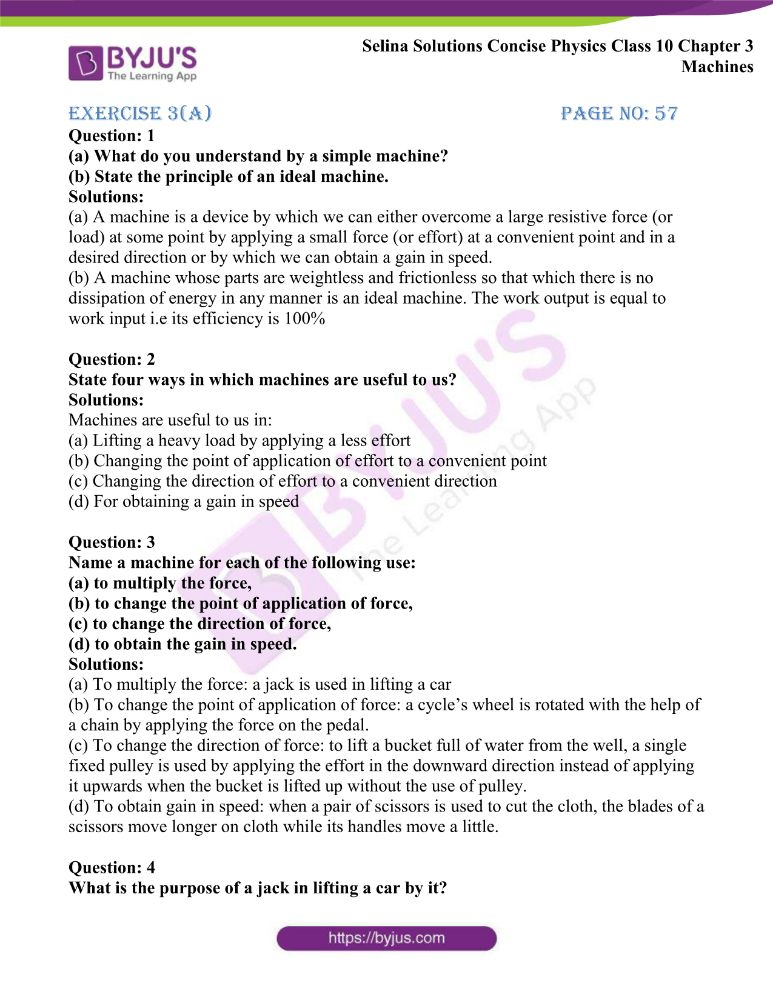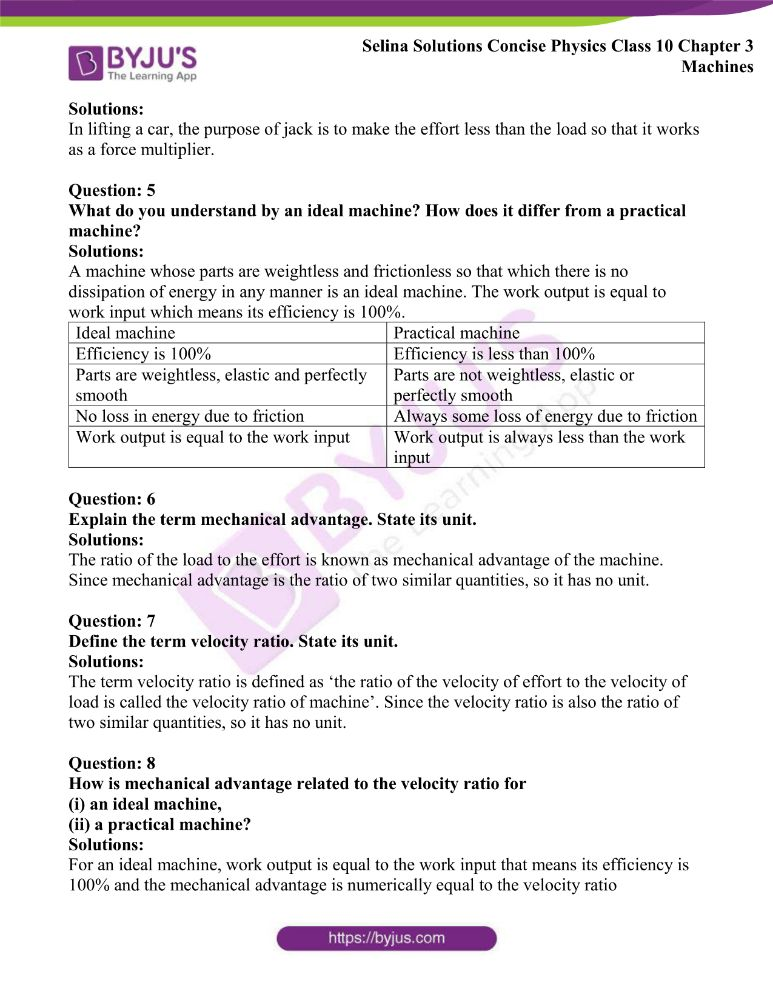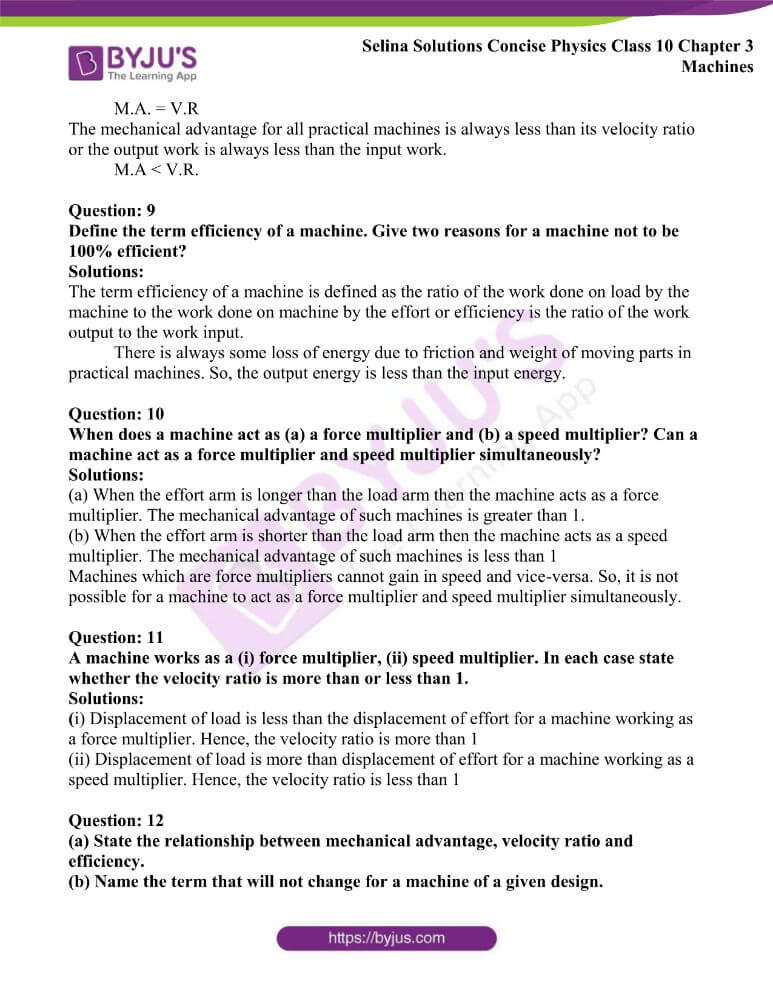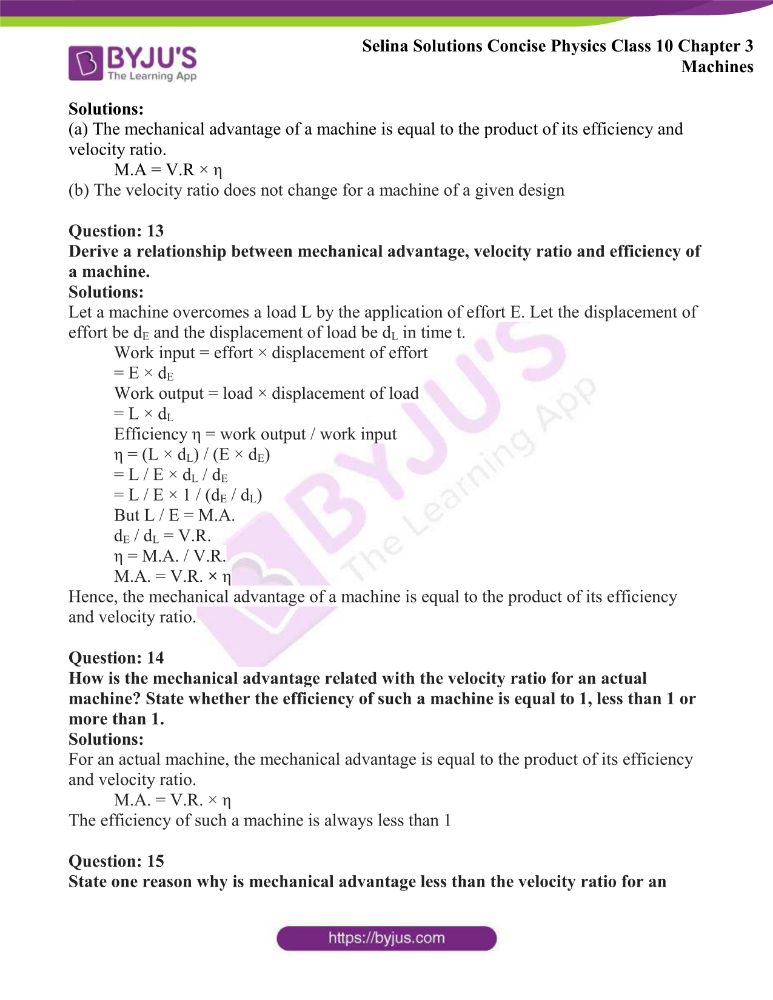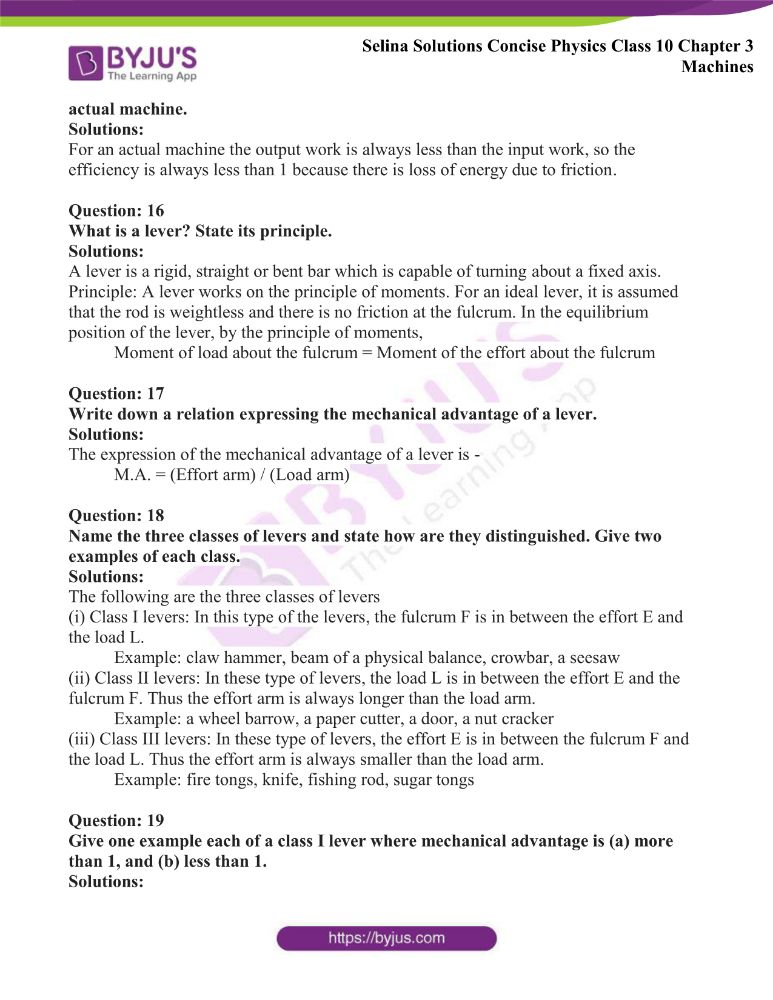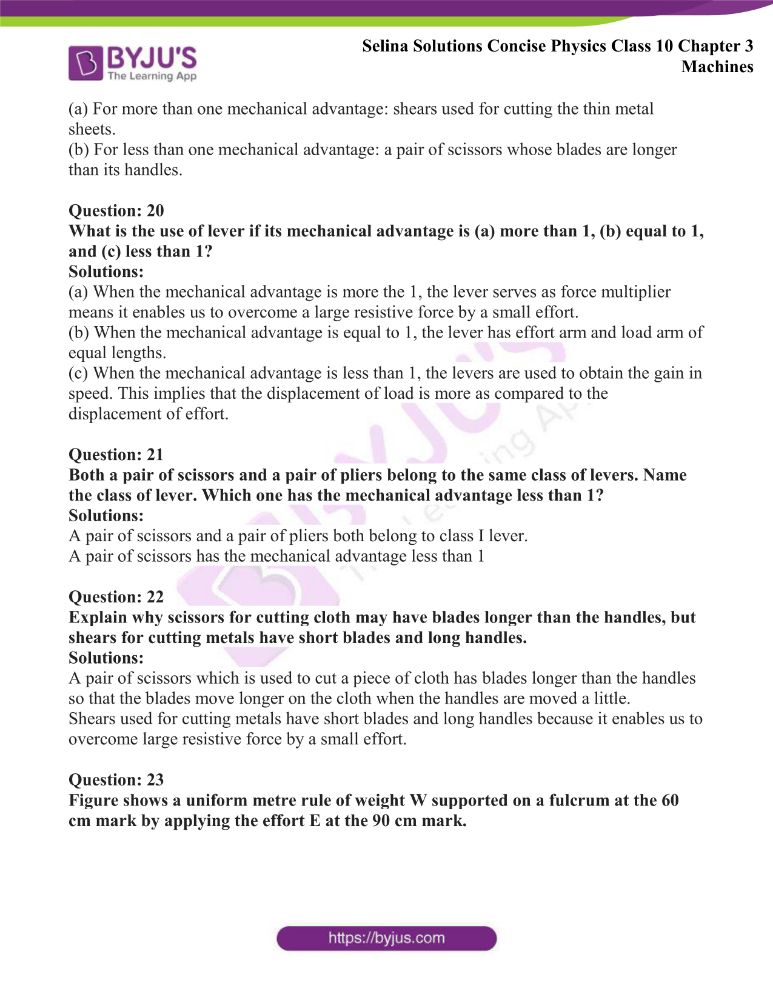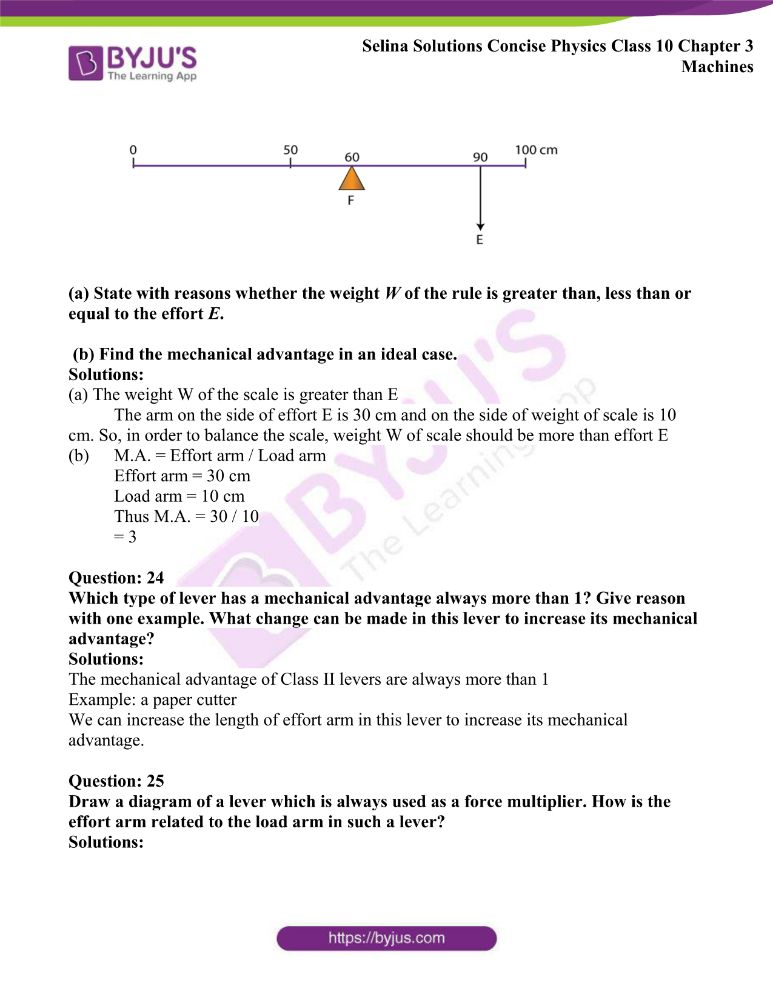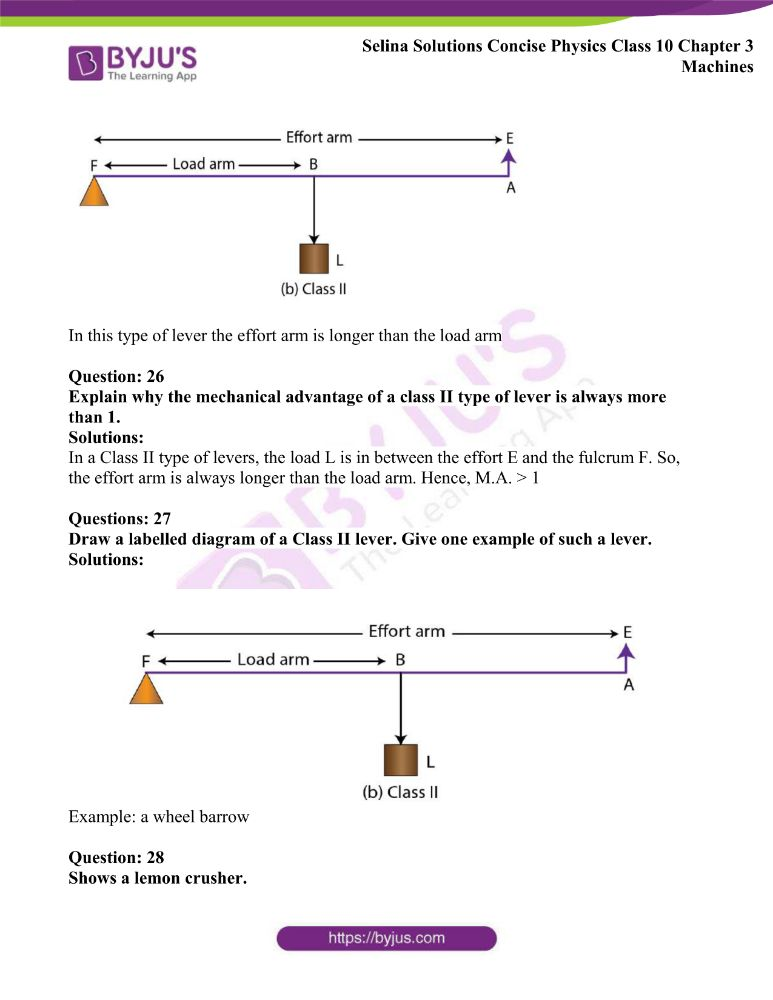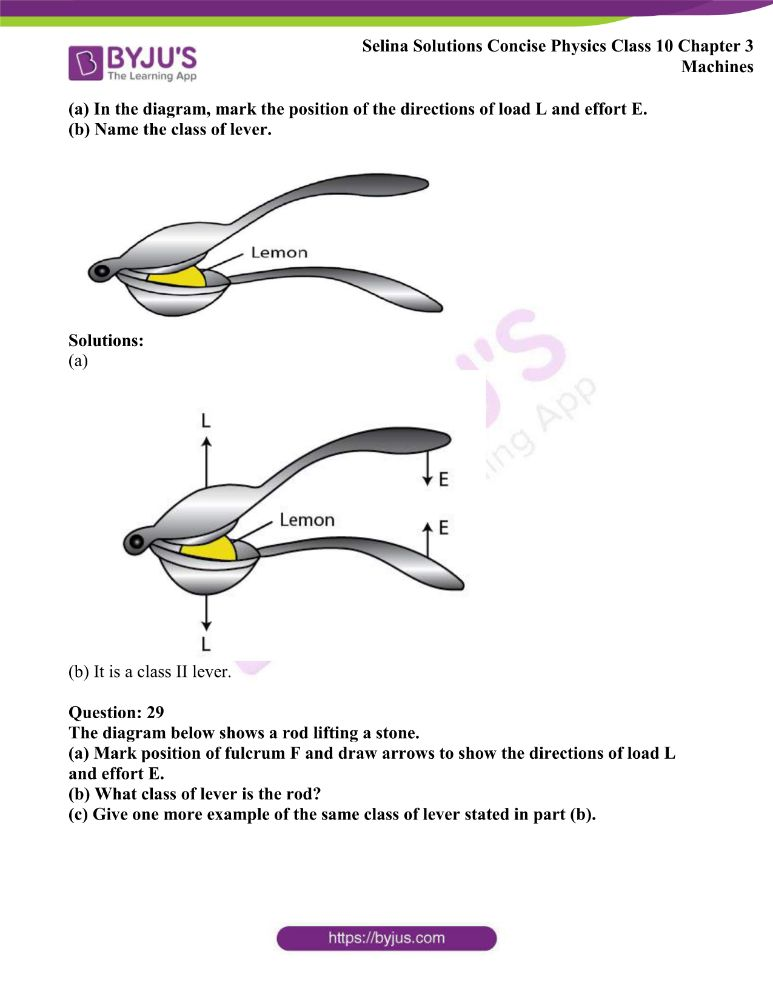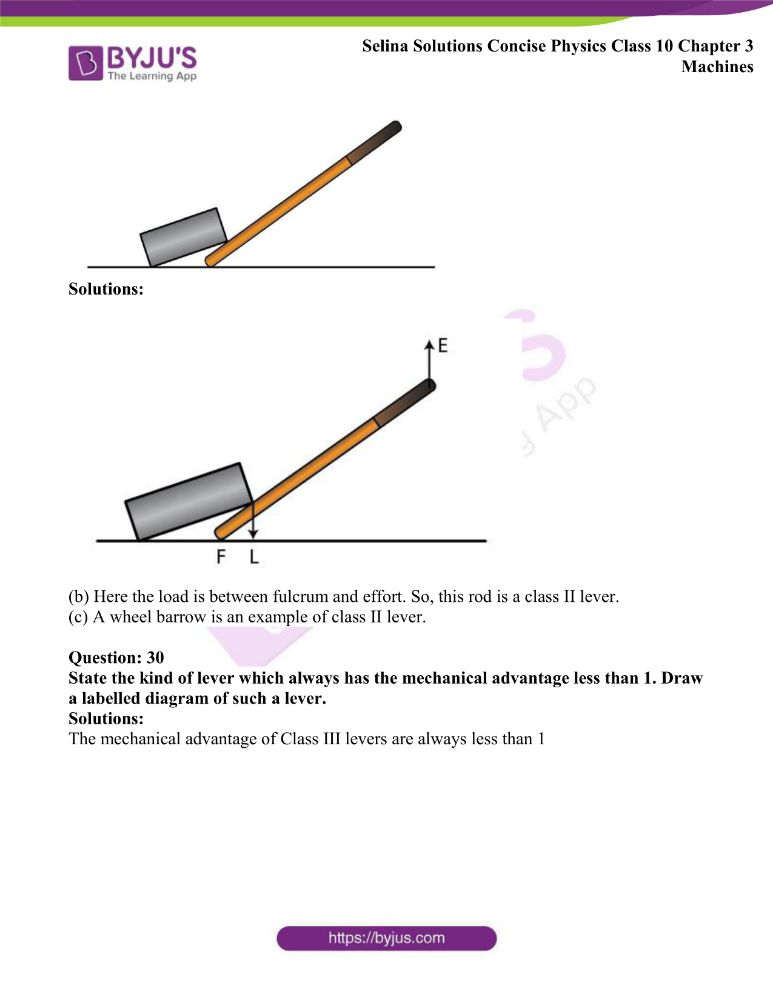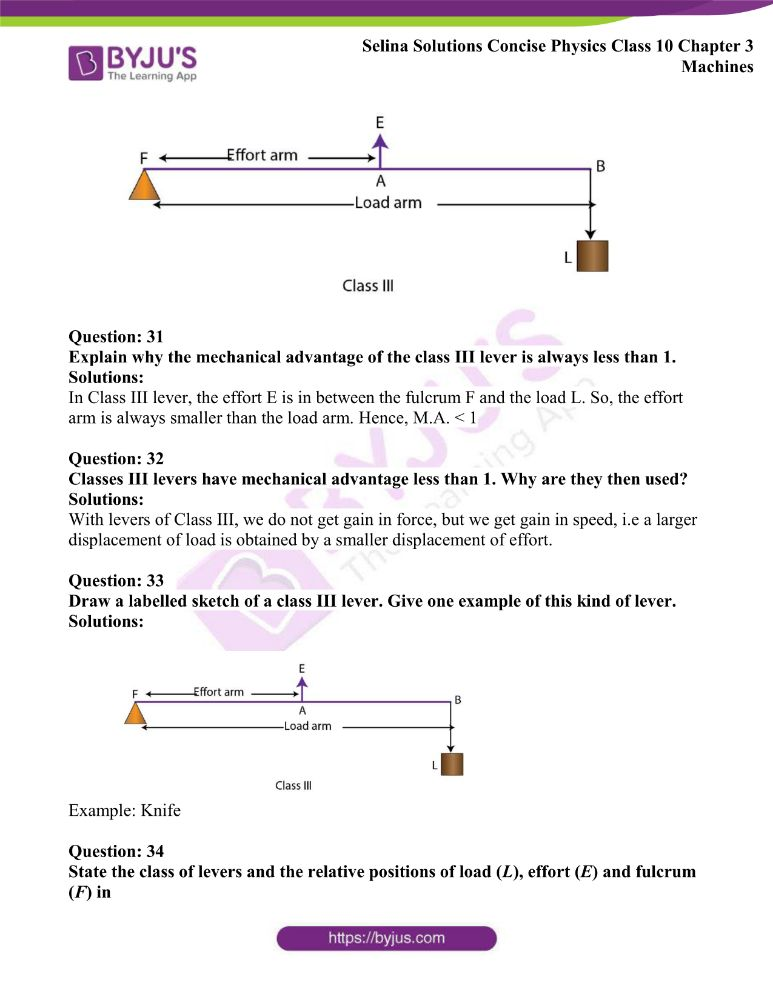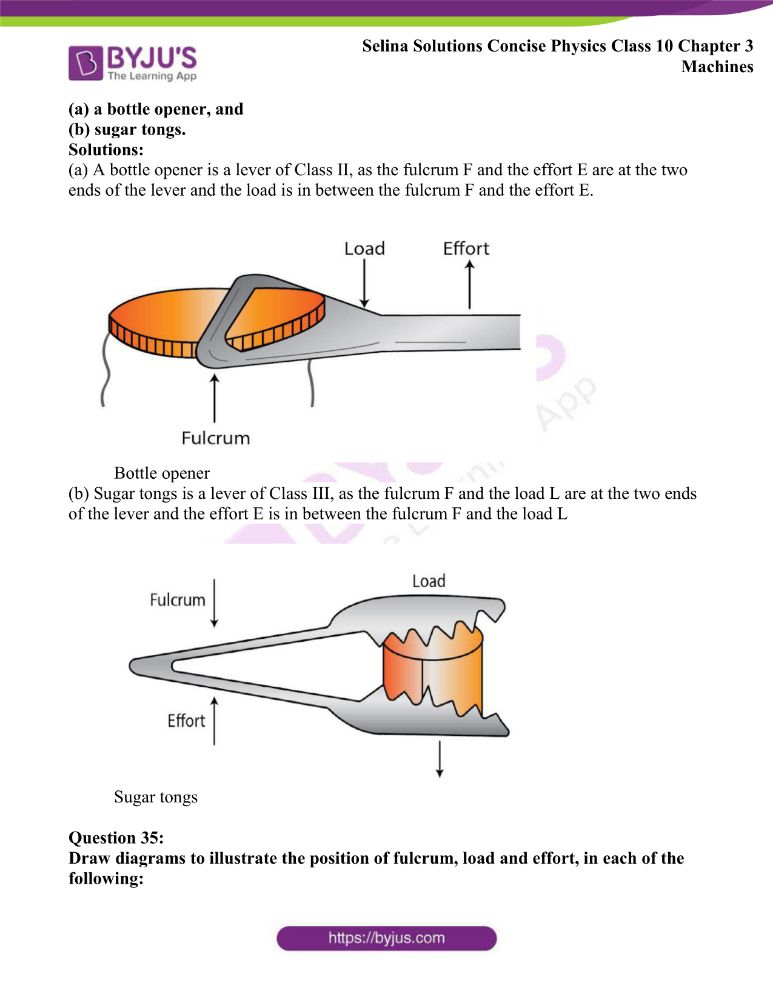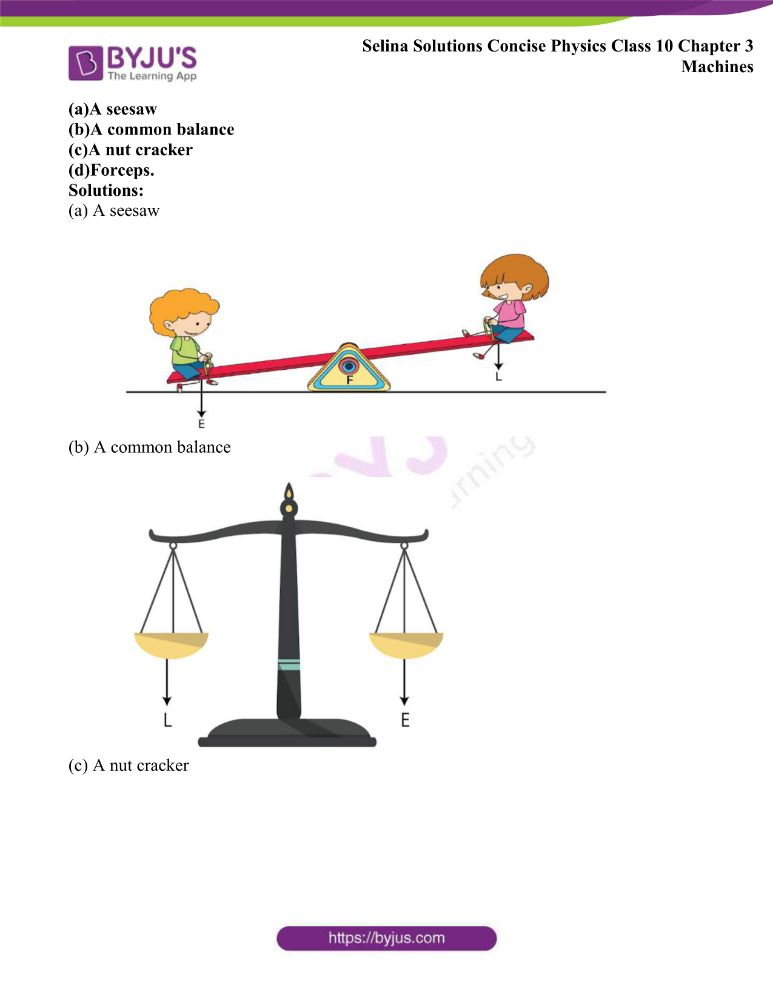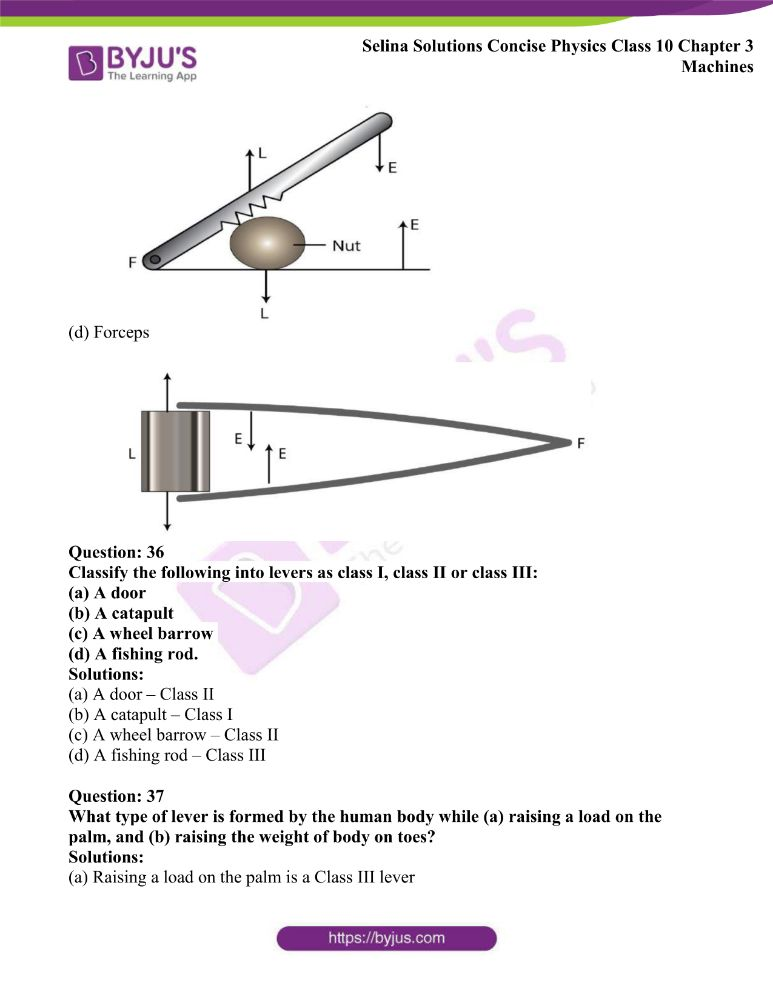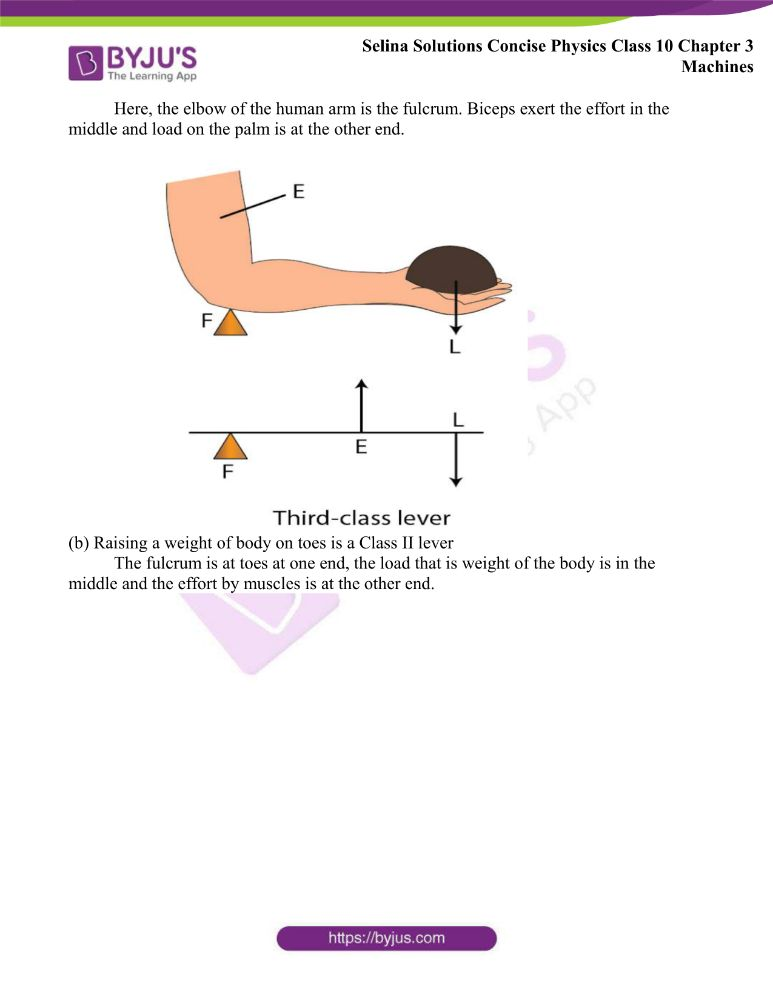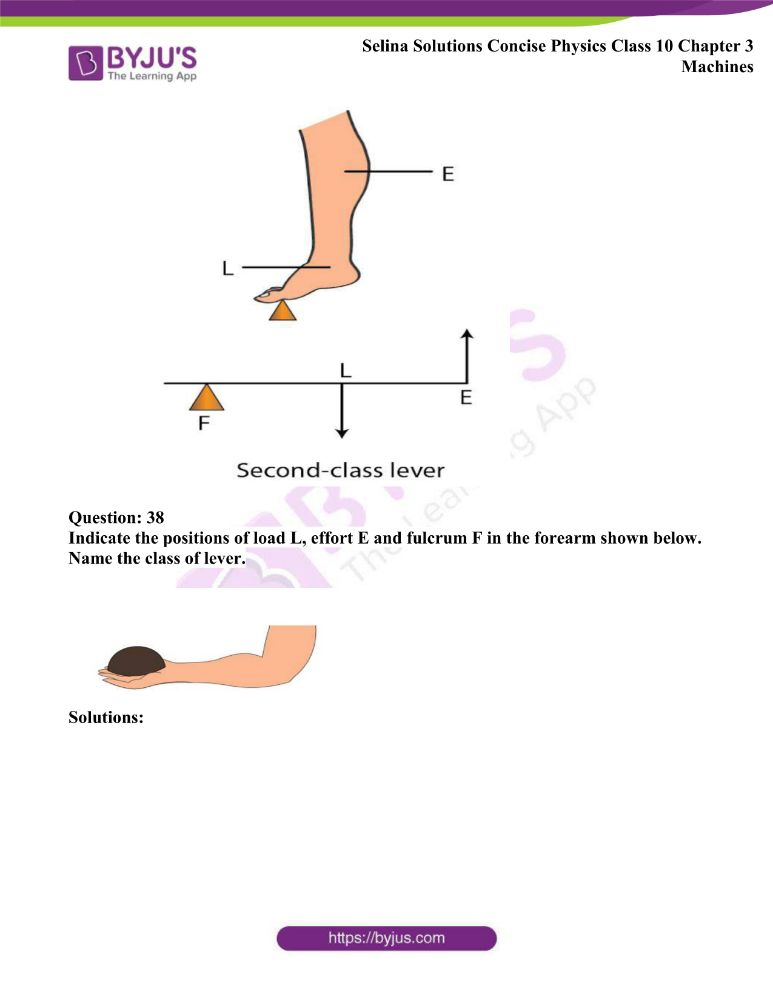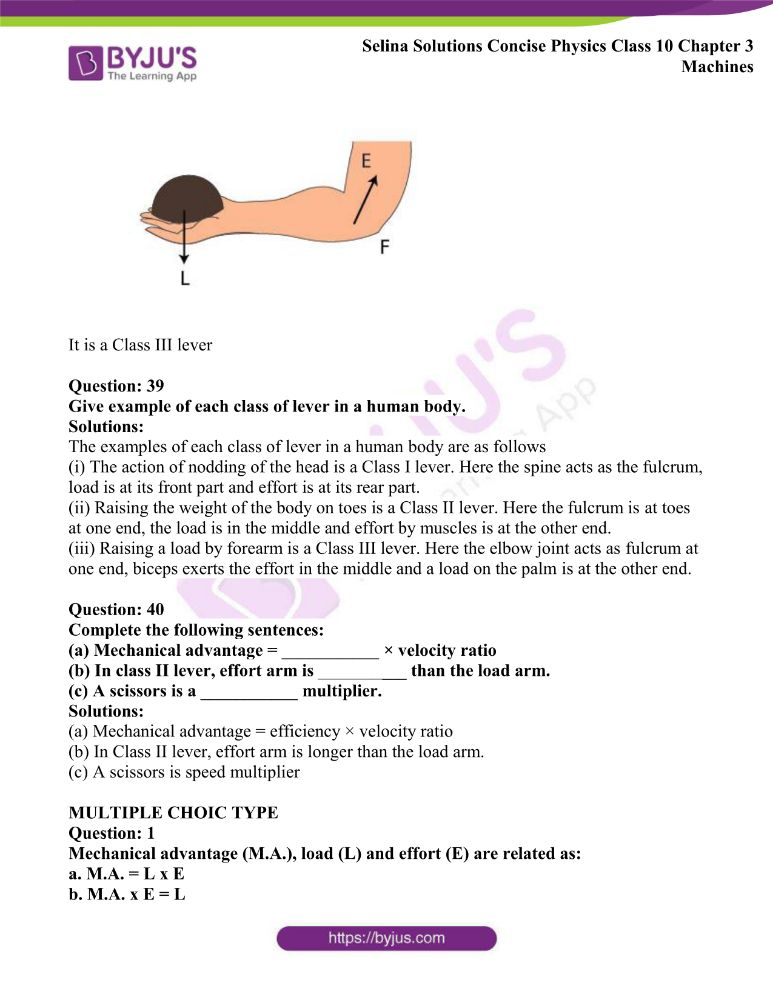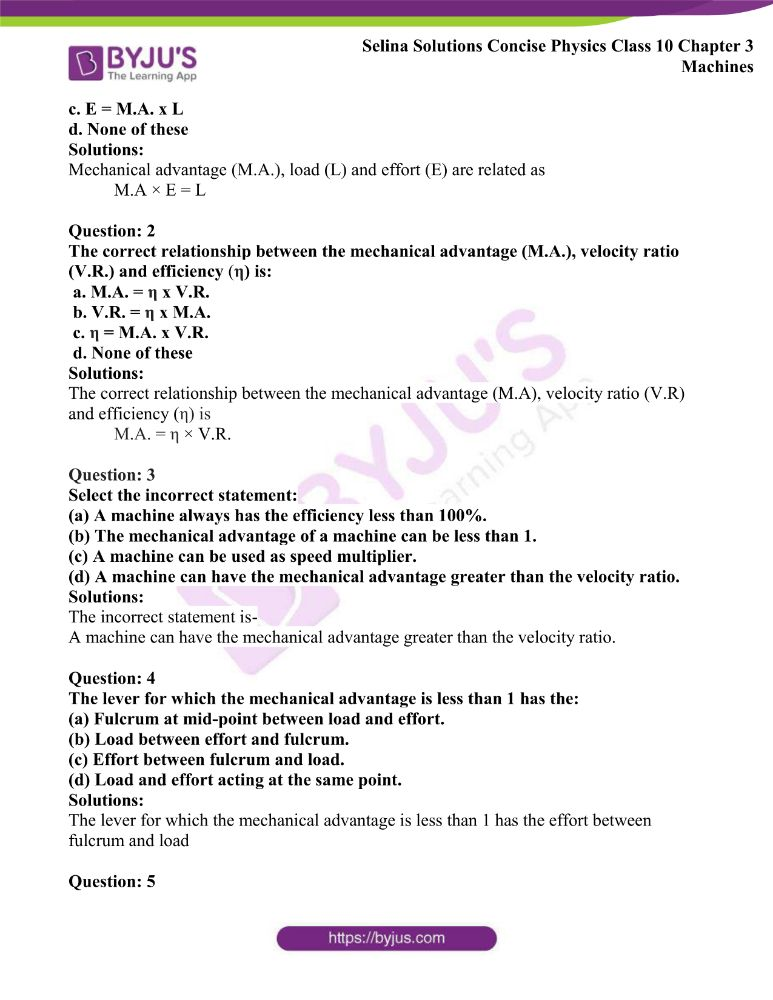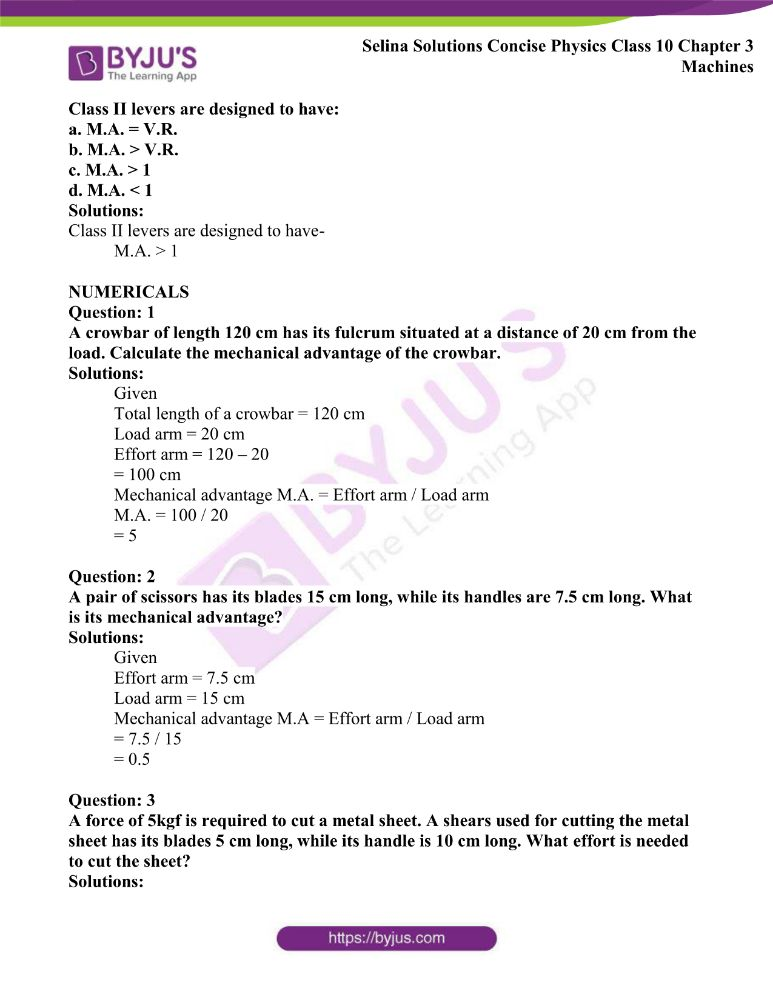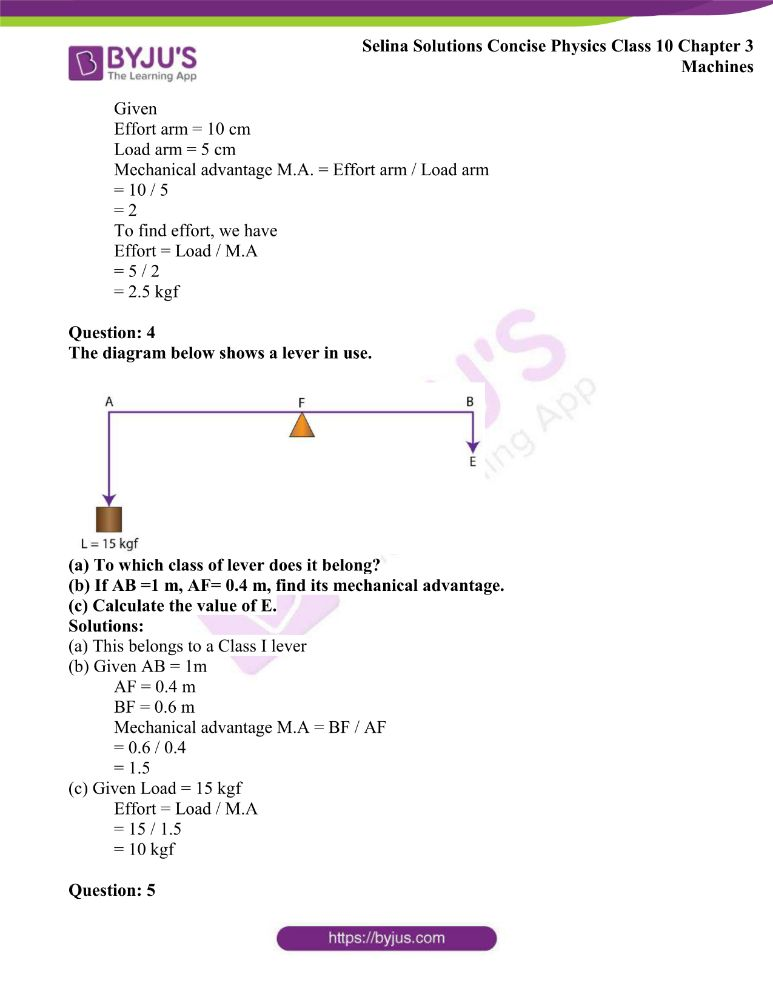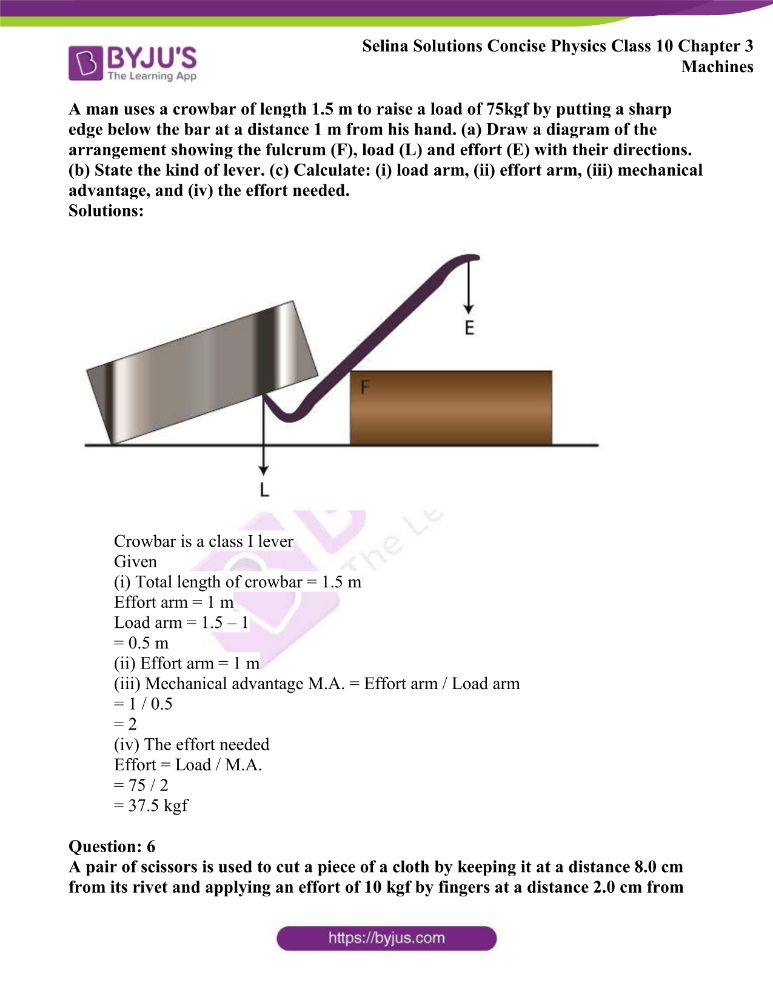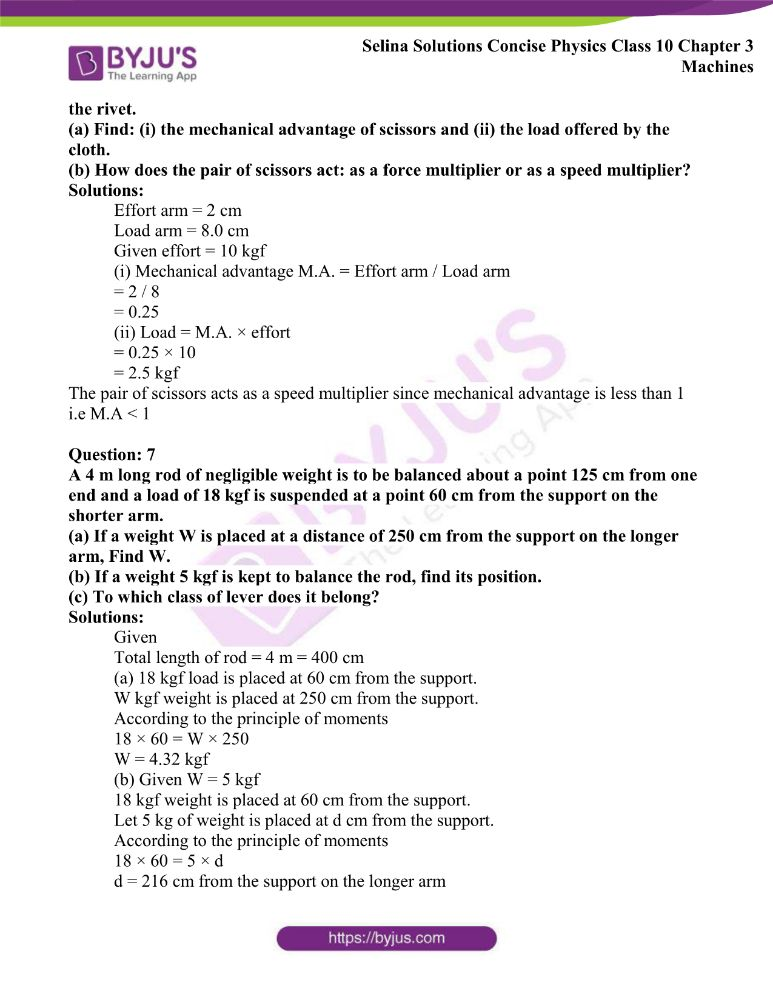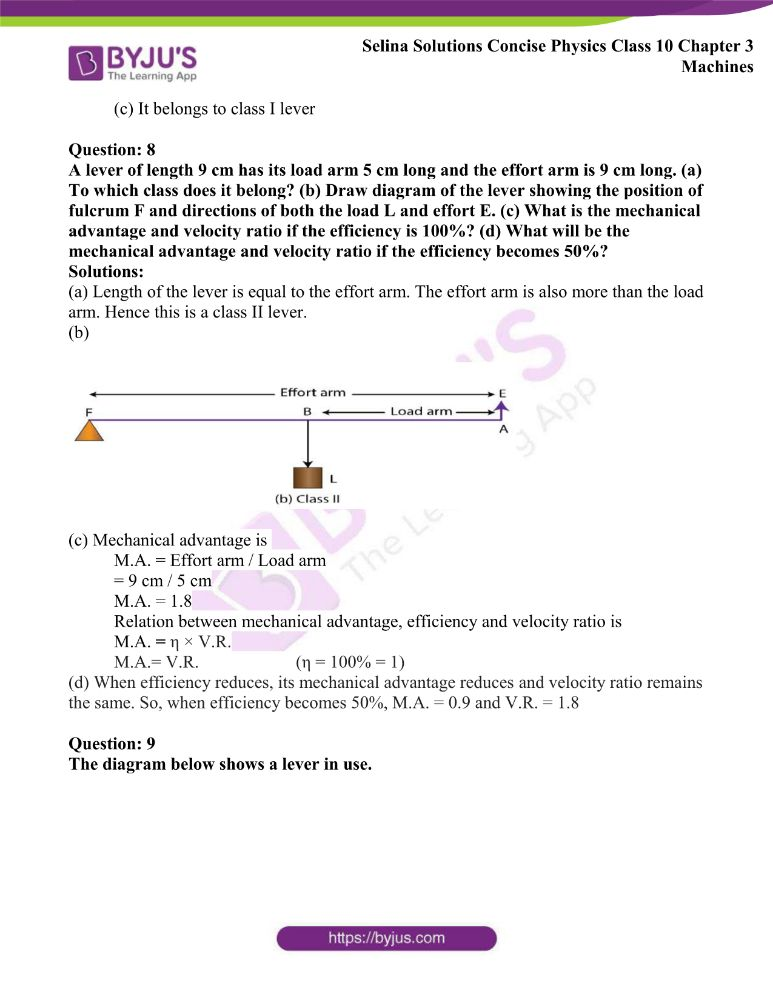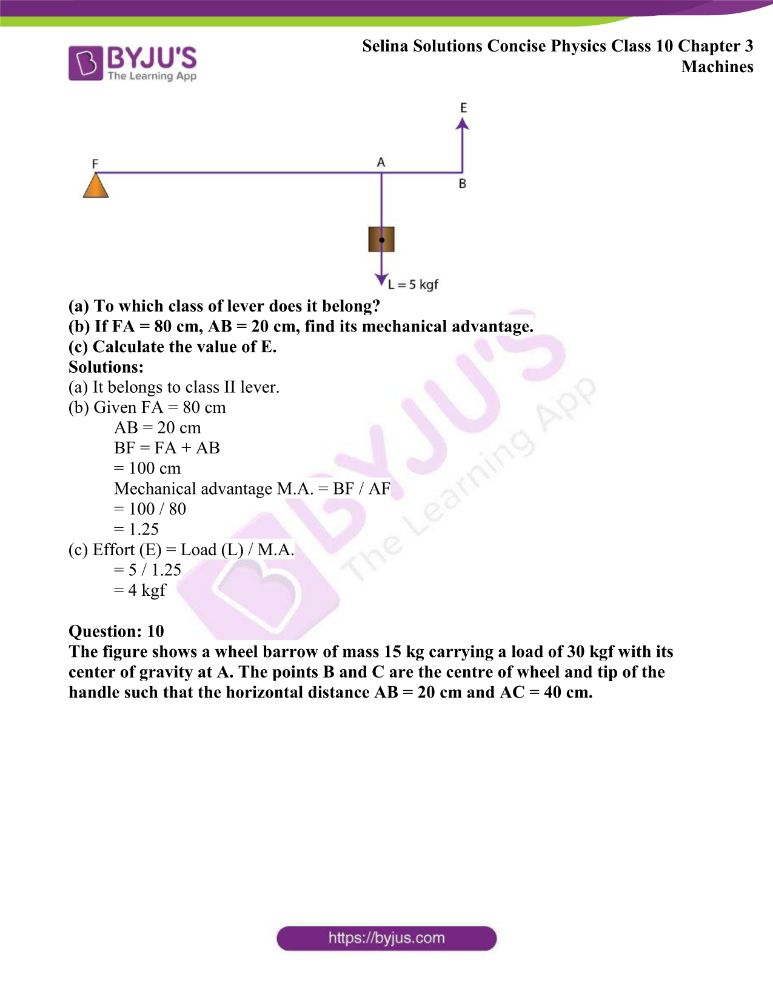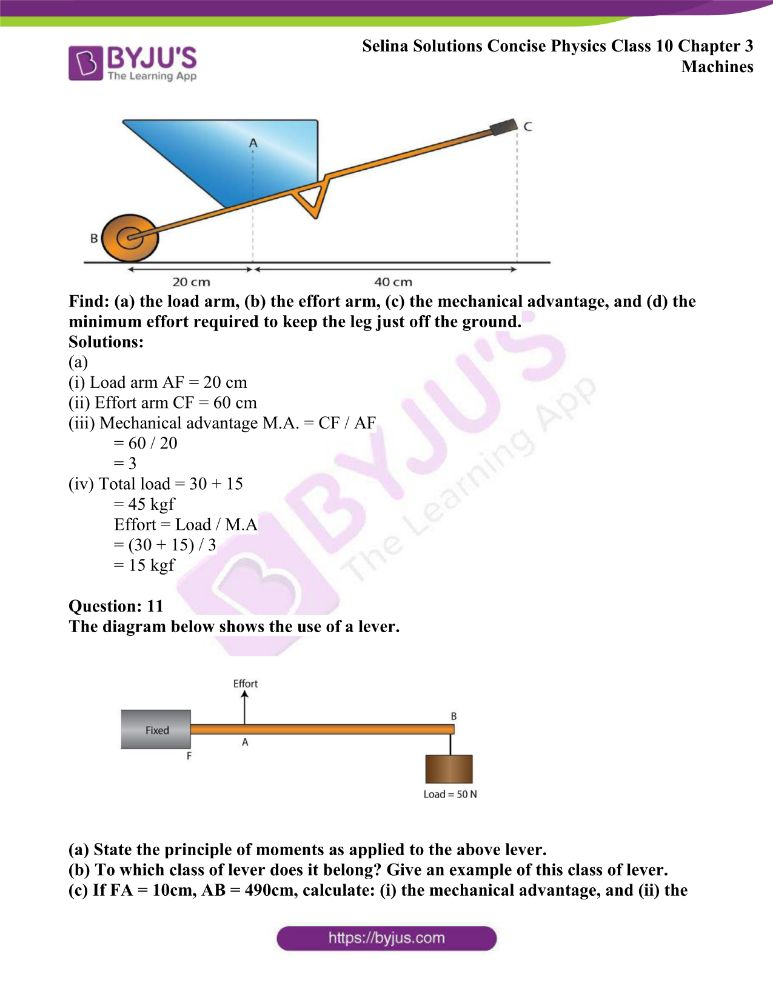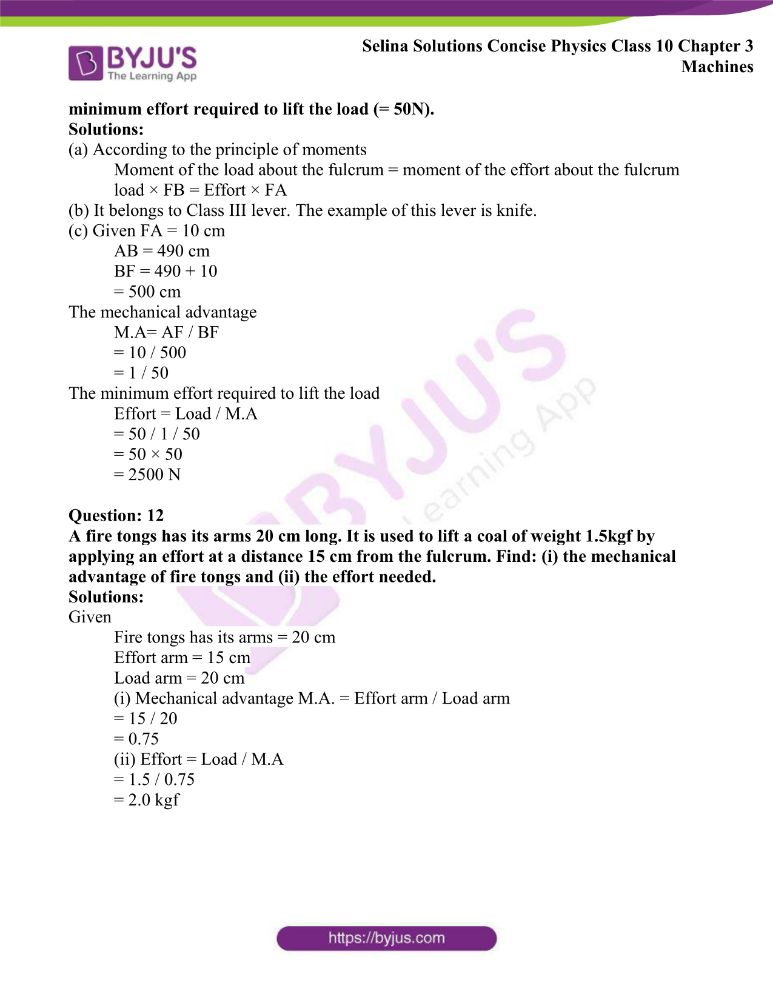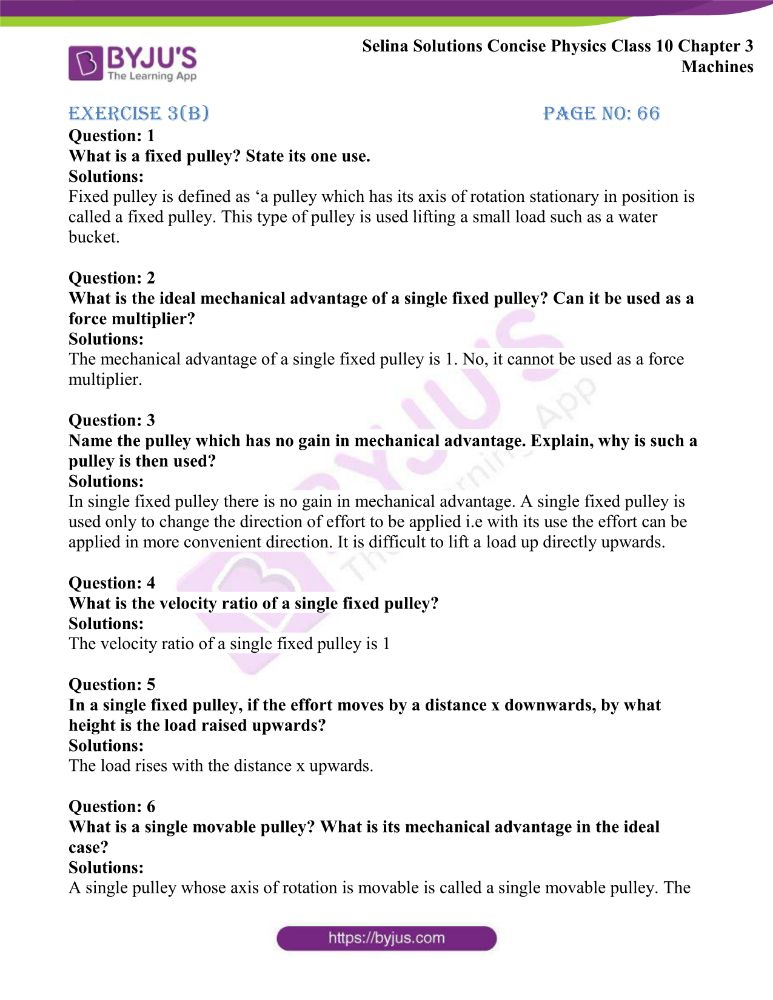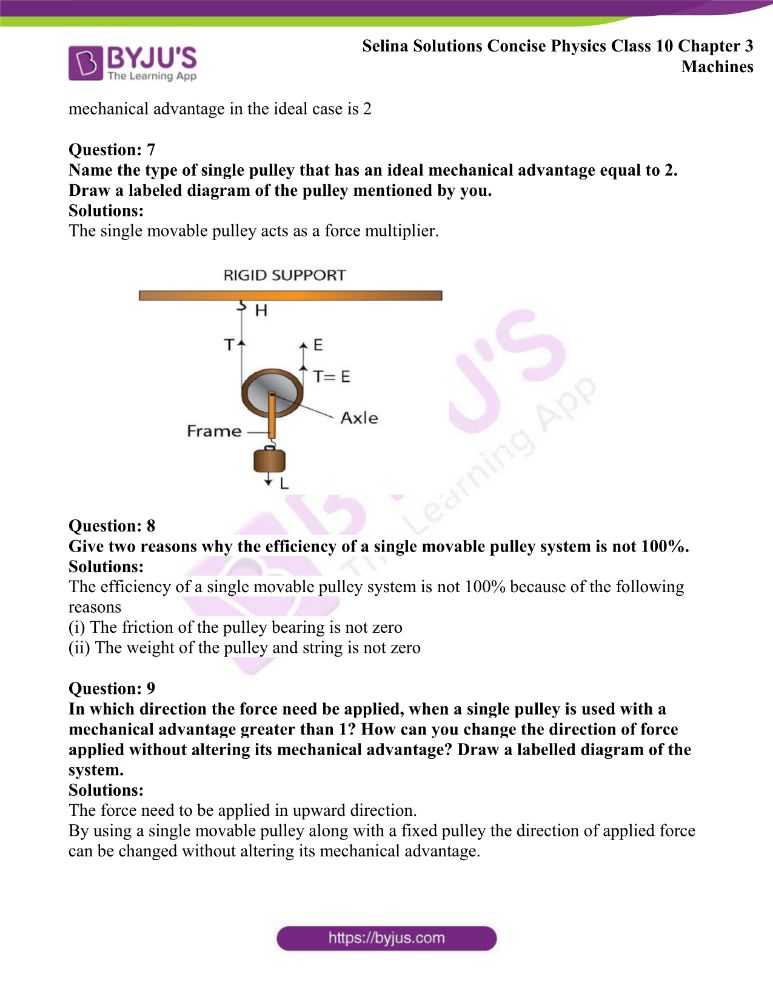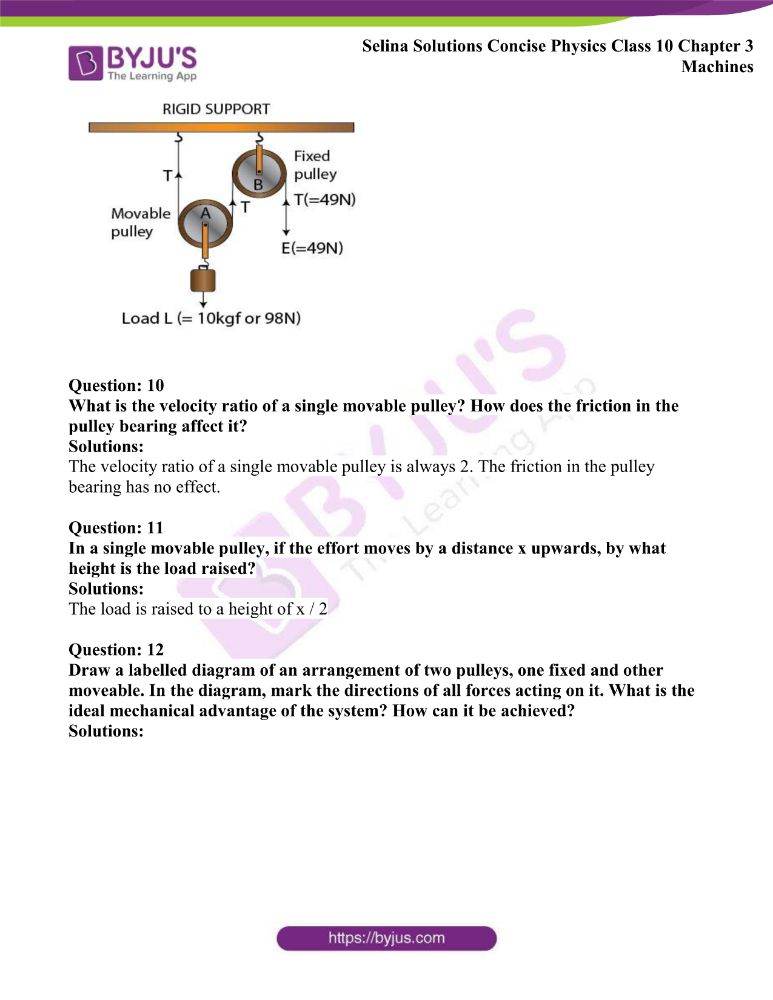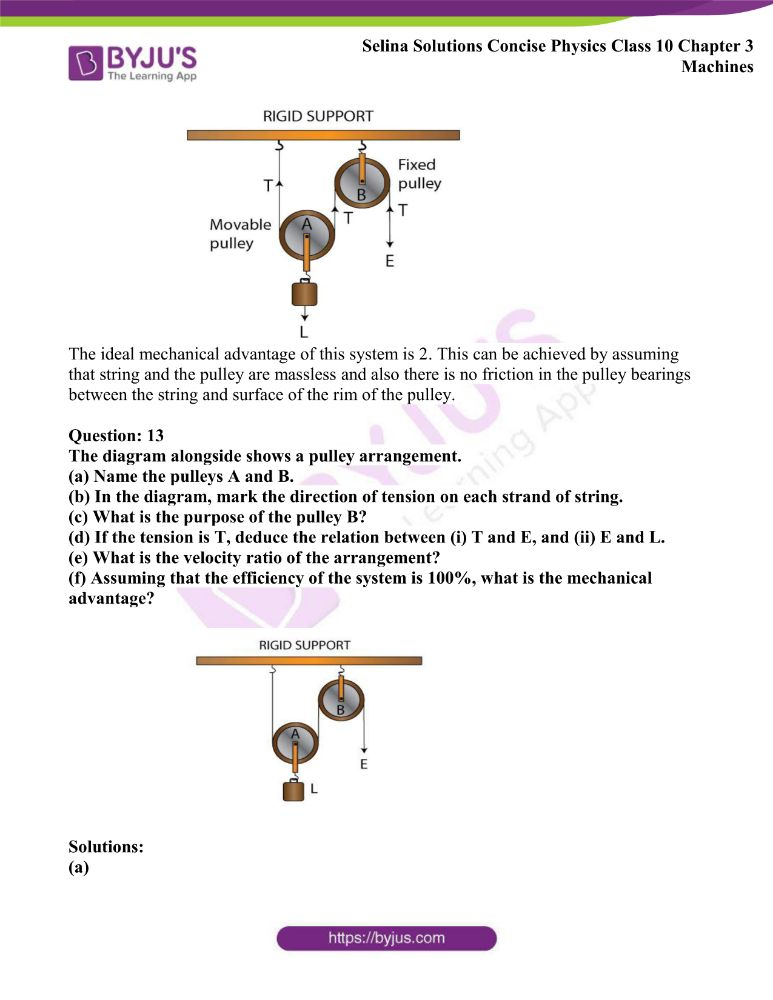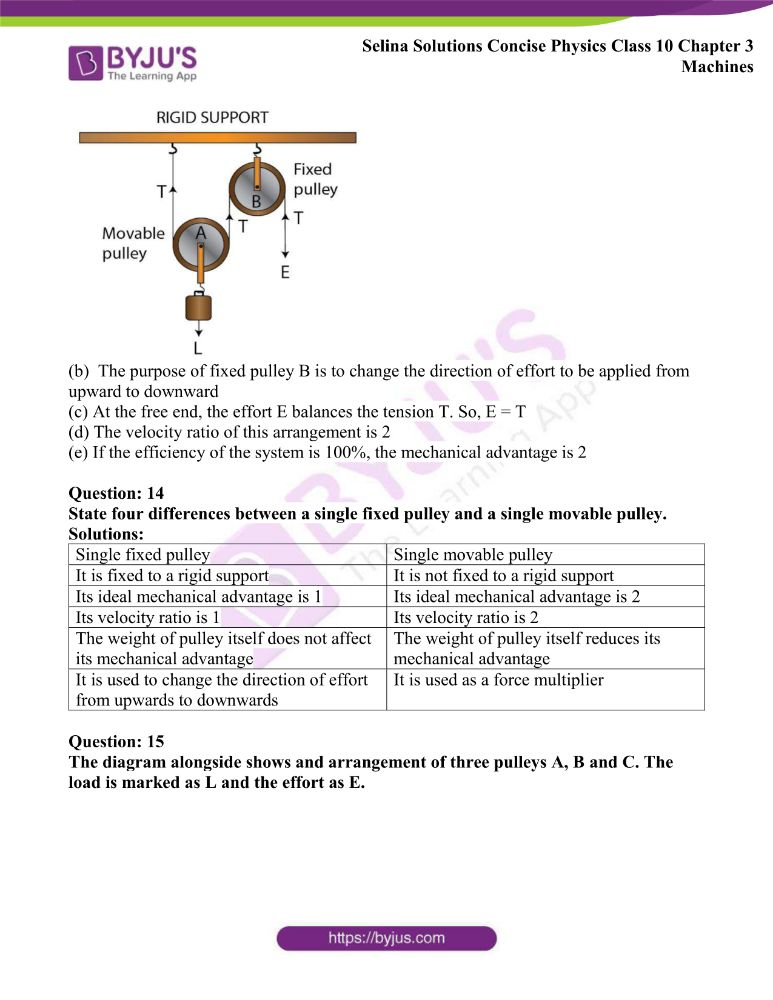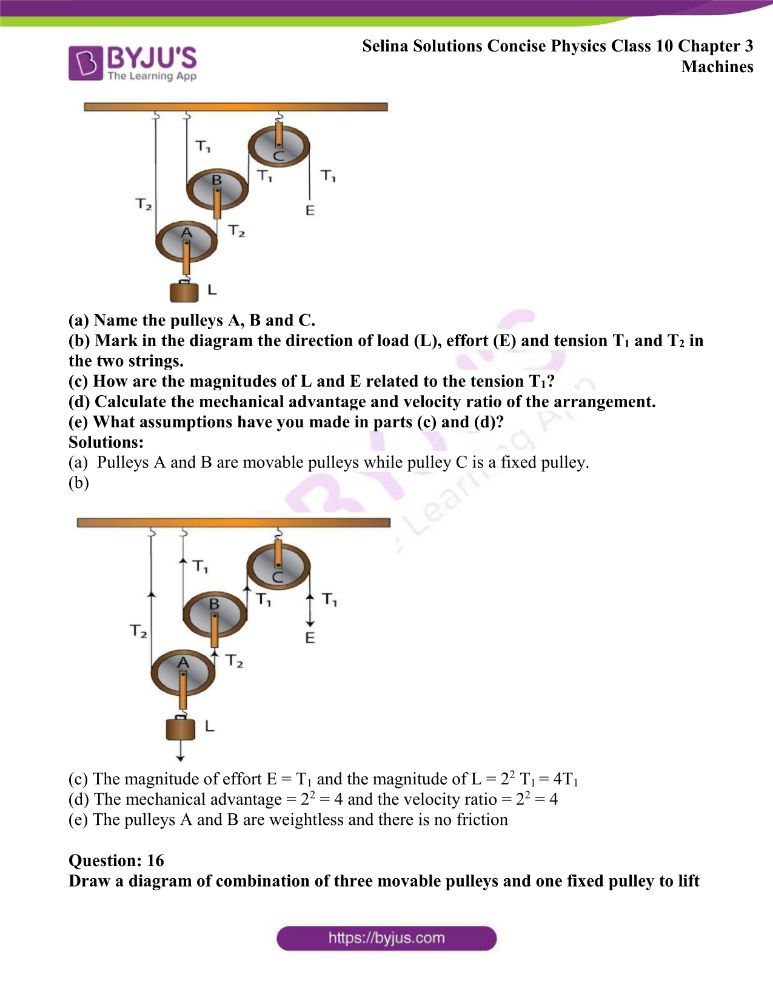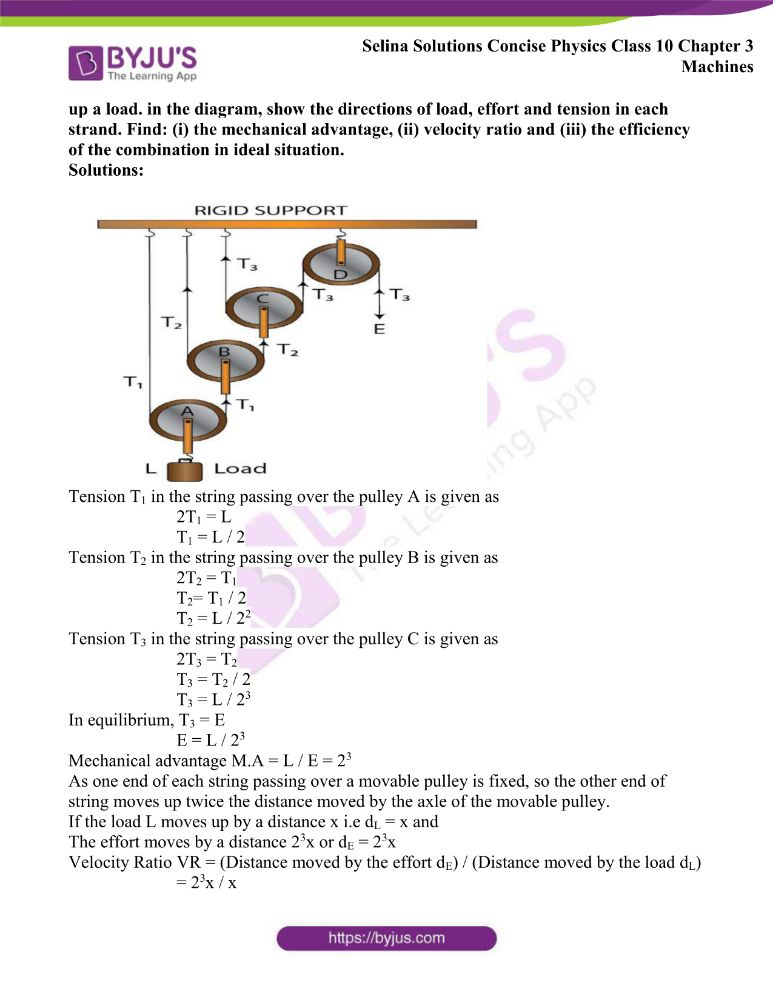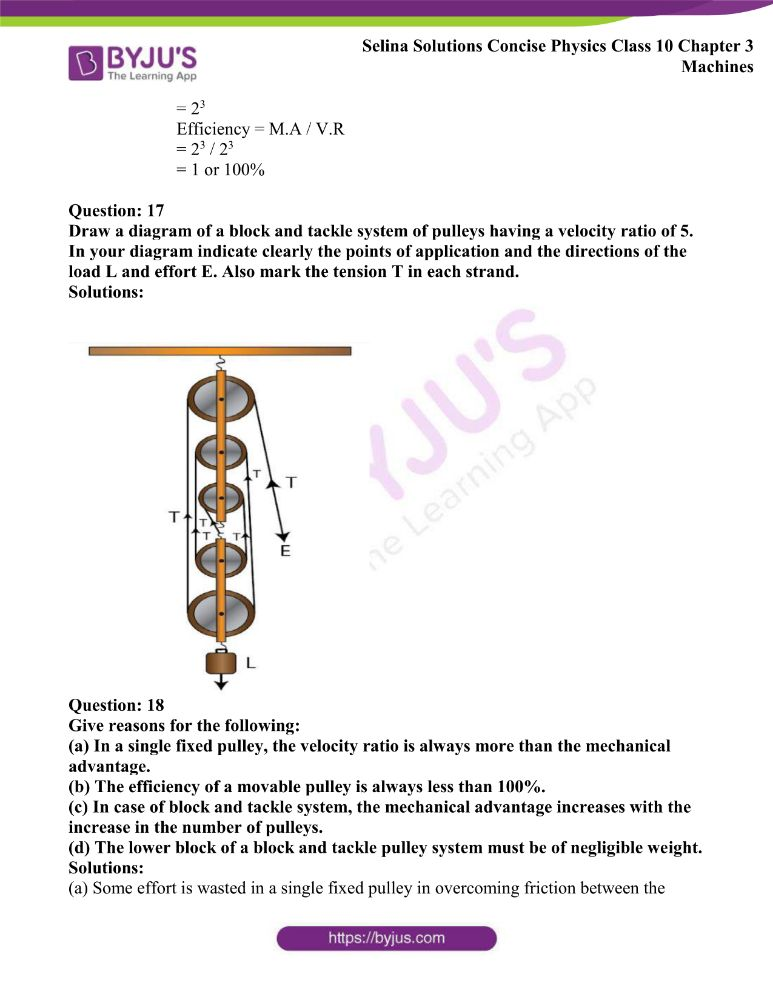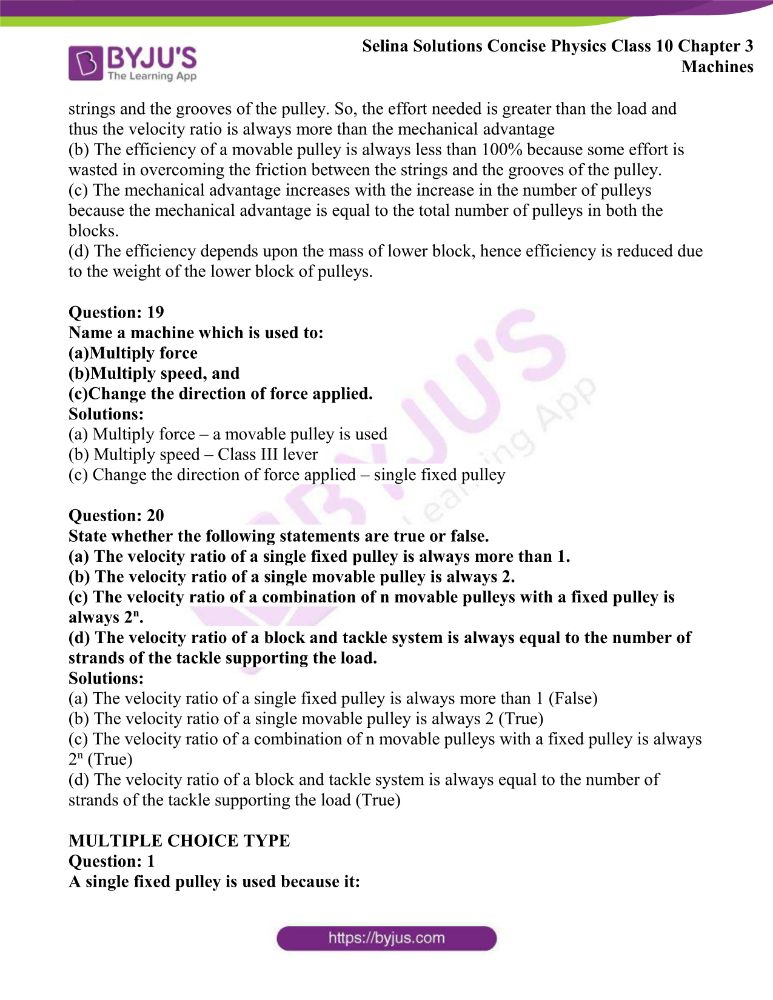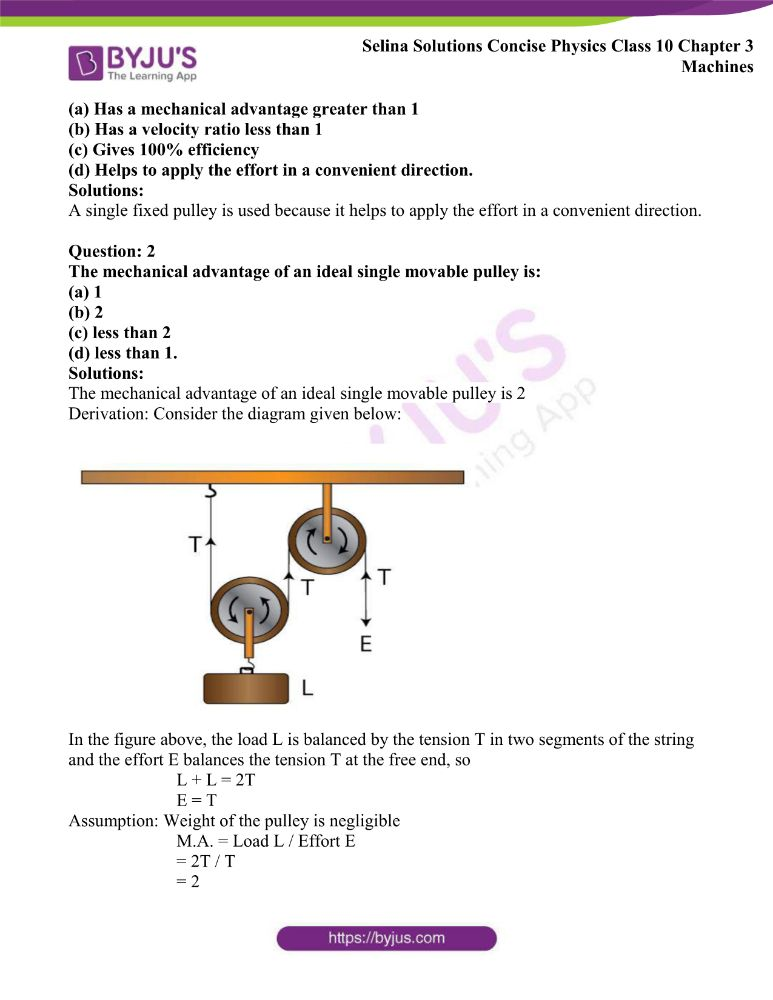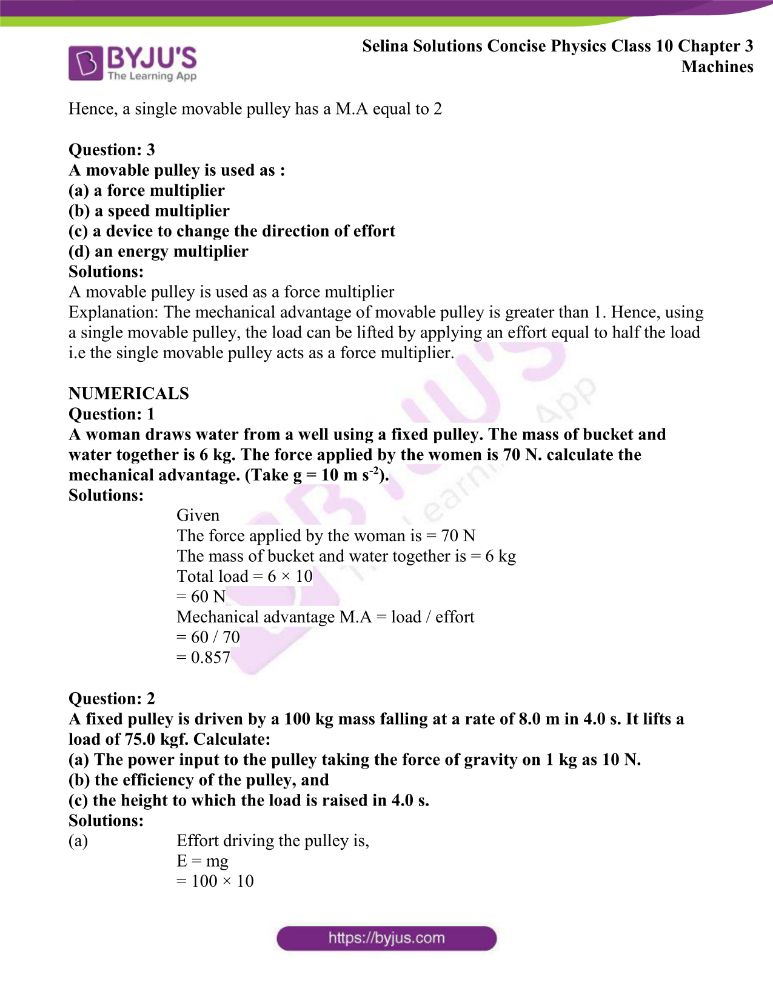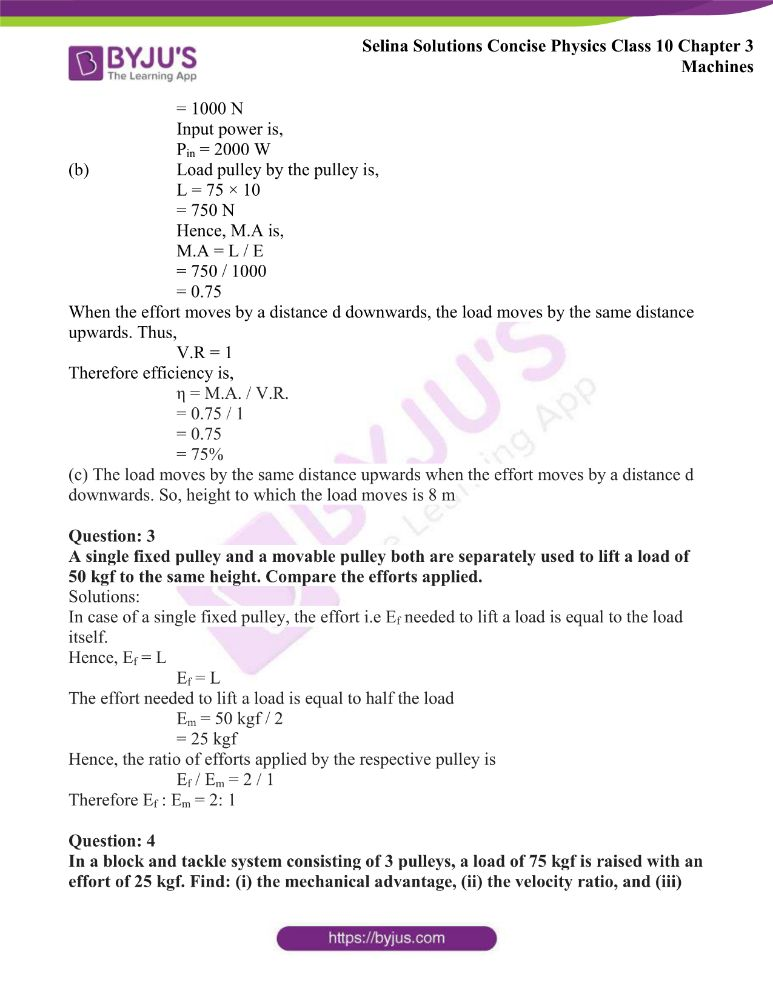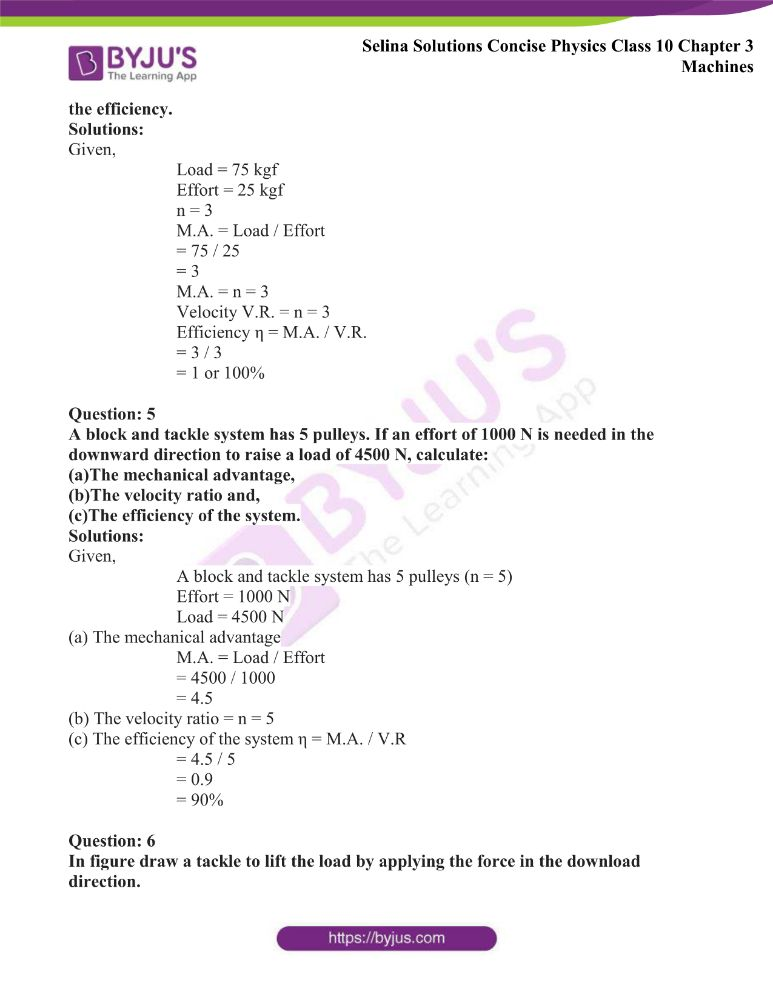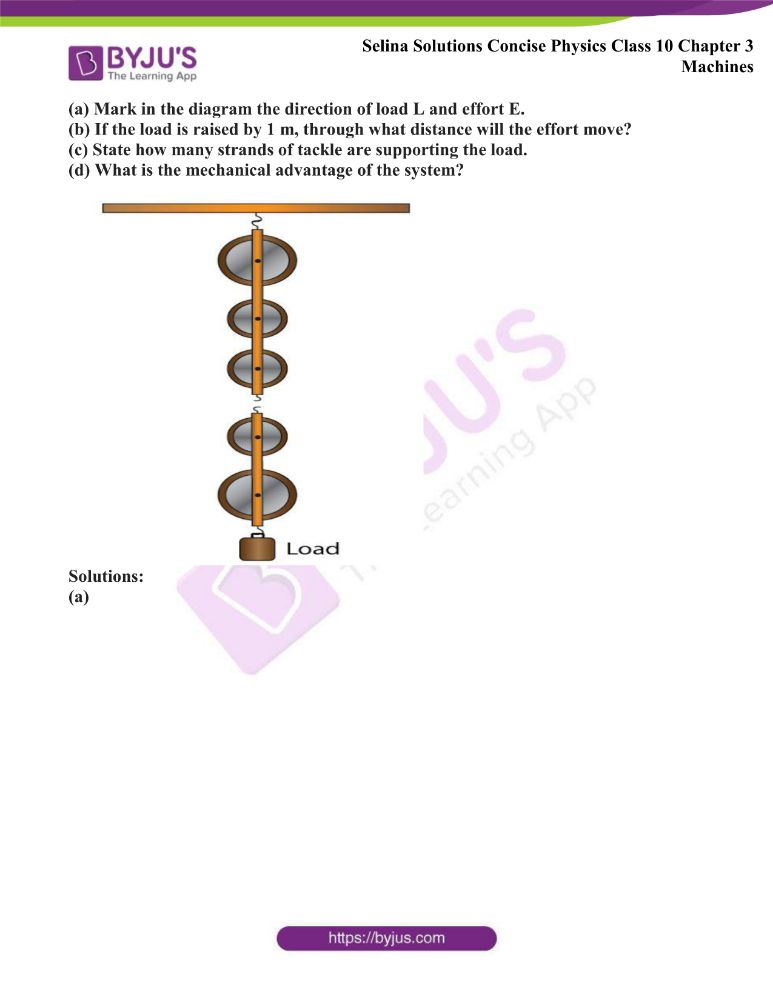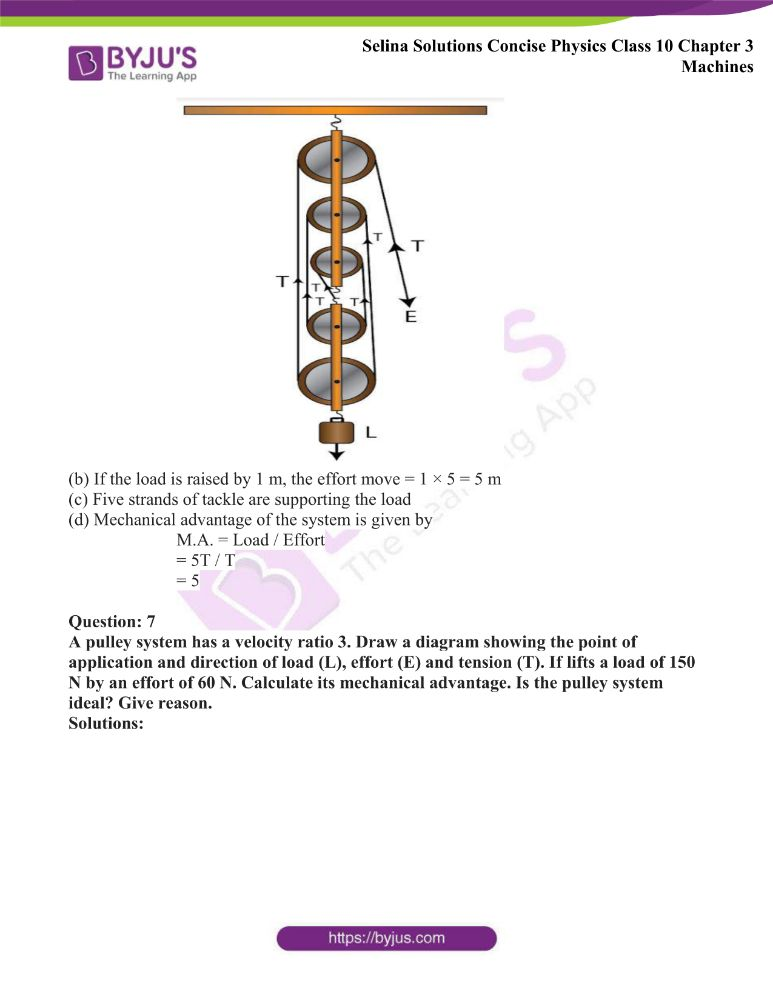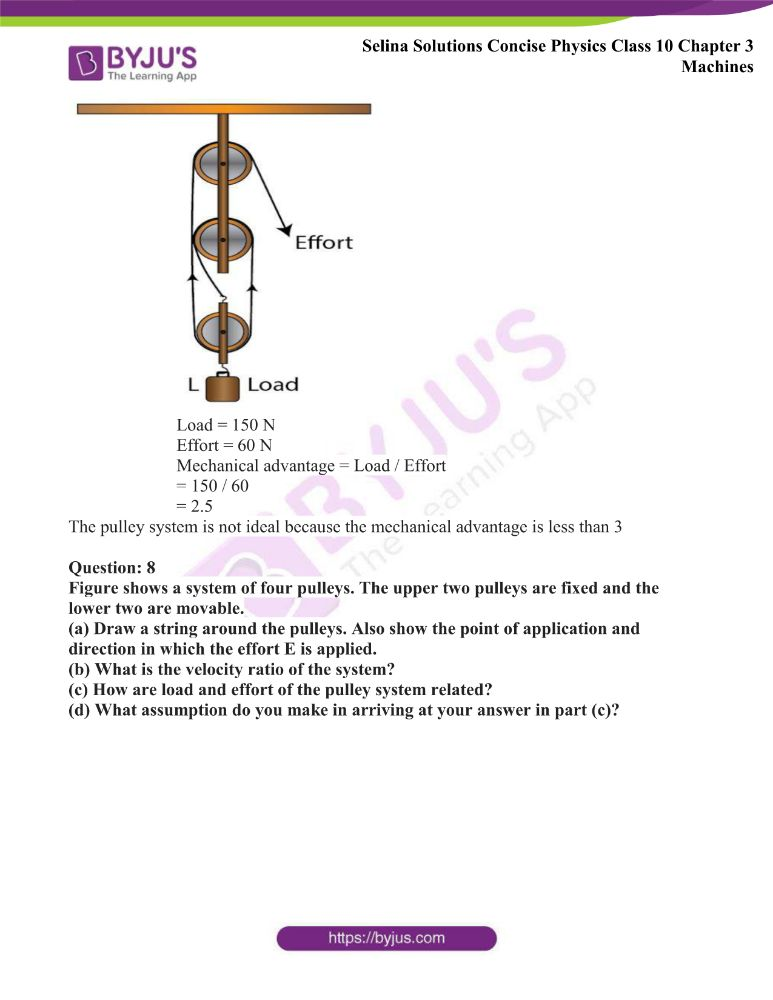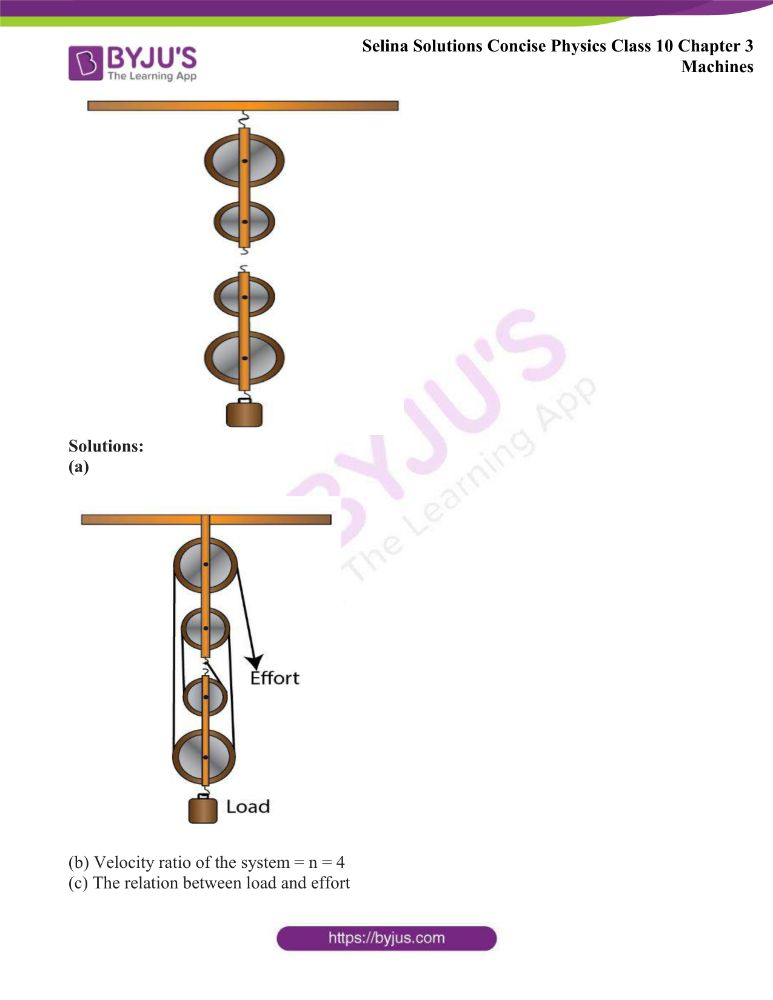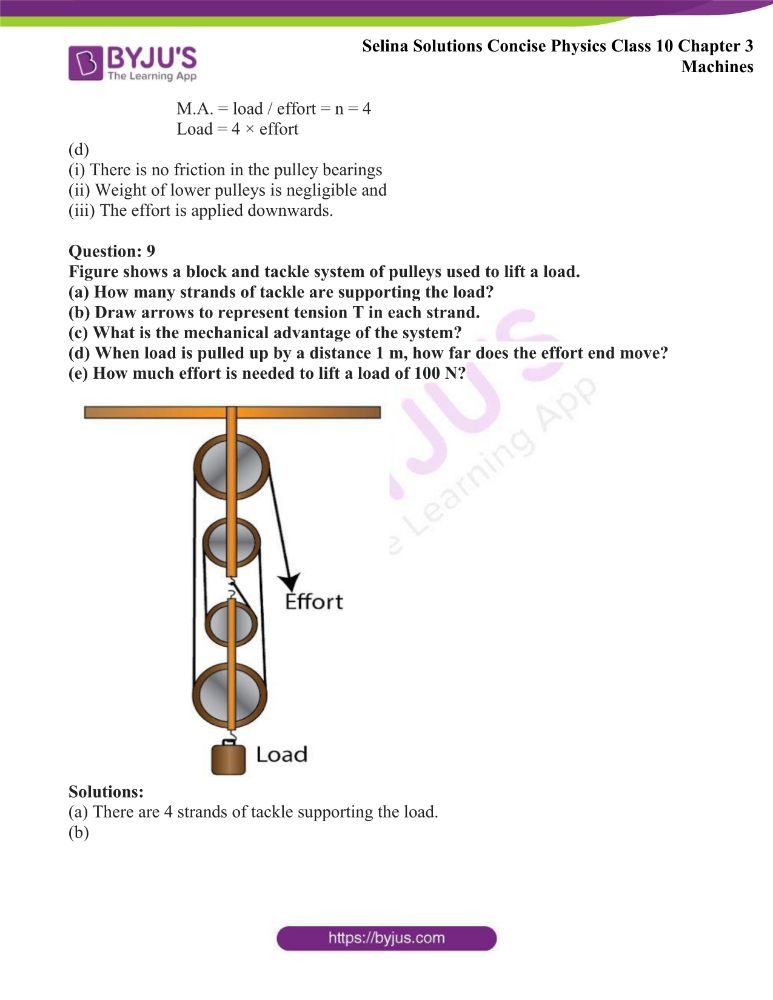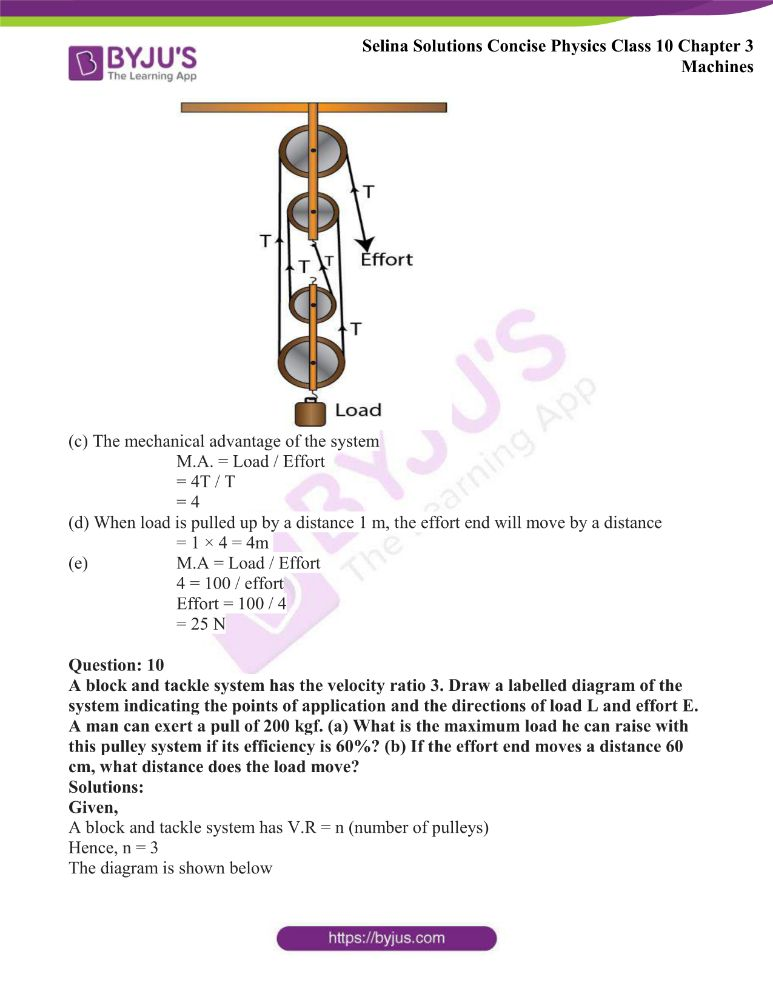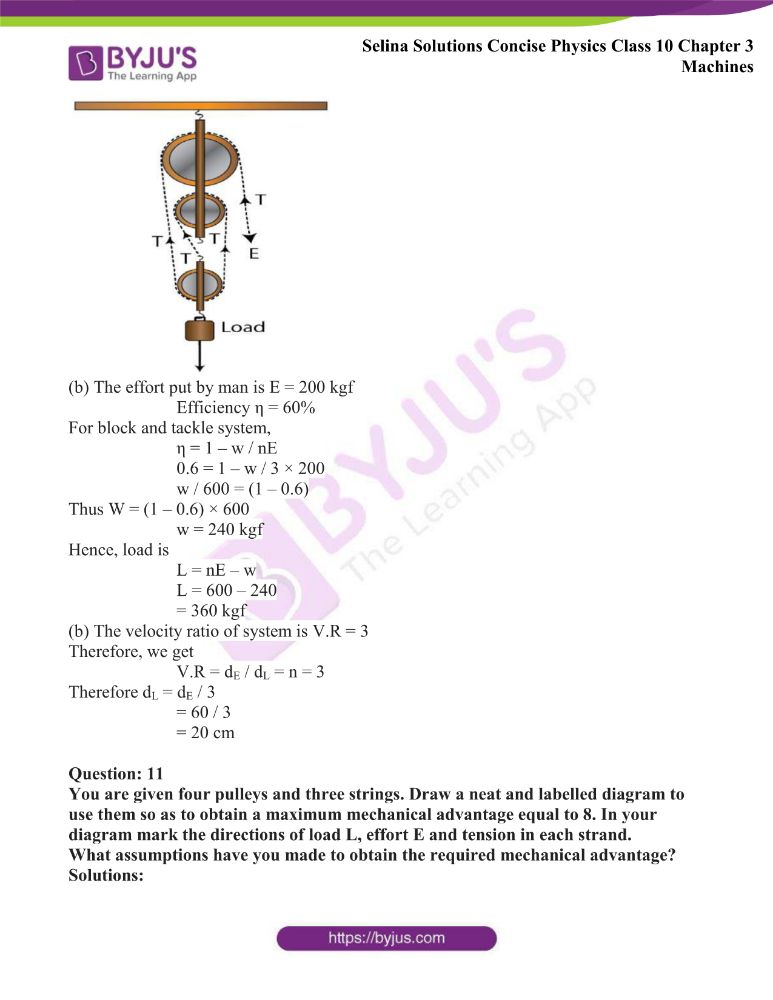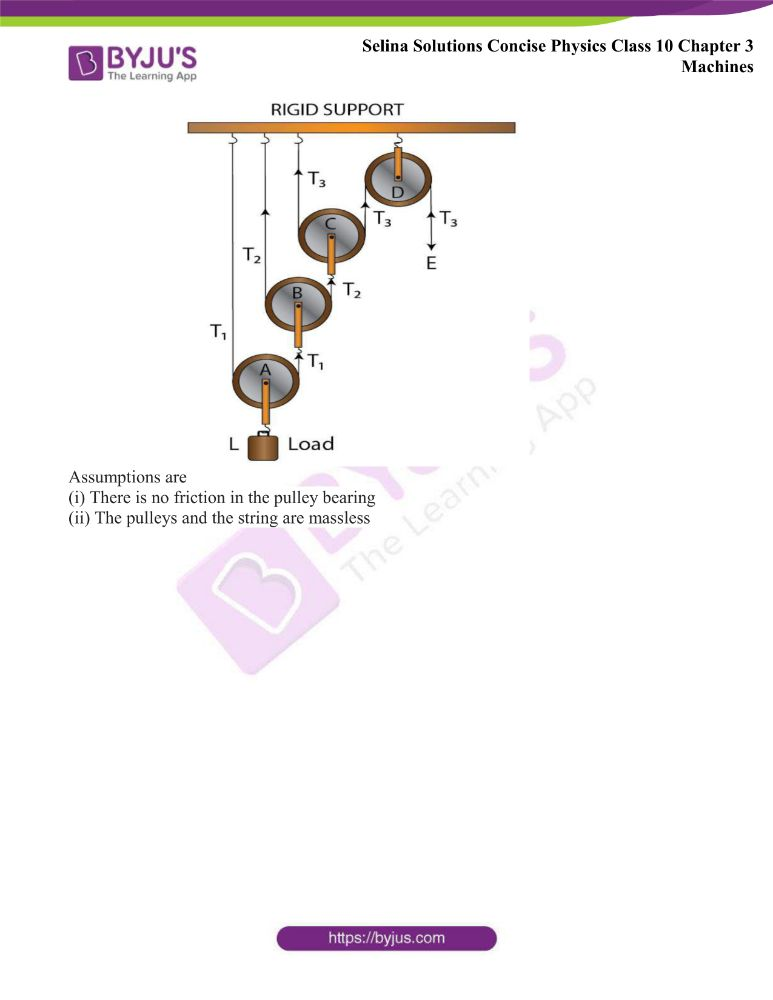### Access Answers of Physics Selina Solutions Concise Physics Class 10 Chapter 3 Machines

Exercise 3(A) page no: 57

Question: 1

(a) What do you understand by a simple machine?

(b) State the principle of an ideal machine.

Solutions:

(a) A machine is a device by which we can either overcome a large resistive force (or load) at some point by applying a small force (or effort) at a convenient point and in a desired direction or by which we can obtain a gain in speed.

(b) A machine whose parts are weightless and frictionless so that which there is no dissipation of energy in any manner is an ideal machine. The work output is equal to work input i.e its efficiency is 100%

Question: 2

State four ways in which machines are useful to us?

Solutions:

Machines are useful to us in:

(a) Lifting a heavy load by applying a less effort

(b) Changing the point of application of effort to a convenient point

(c) Changing the direction of effort to a convenient direction

(d) For obtaining a gain in speed

Question: 3

Name a machine for each of the following use:

(a) to multiply the force,

(b) to change the point of application of force,

(c) to change the direction of force,

(d) to obtain the gain in speed.

Solutions:

(a) To multiply the force: a jack is used in lifting a car

(b) To change the point of application of force: a cycle’s wheel is rotated with the help of a chain by applying the force on the pedal.

(c) To change the direction of force: to lift a bucket full of water from the well, a single fixed pulley is used by applying the effort in the downward direction instead of applying it upwards when the bucket is lifted up without the use of pulley.

(d) To obtain gain in speed: when a pair of scissors is used to cut the cloth, the blades of a scissors move longer on cloth while its handles move a little.

Question: 4

What is the purpose of a jack in lifting a car by it?

Solutions:

In lifting a car, the purpose of the jack is to make the effortless than the load so that it works as a force multiplier.

Question: 5

What do you understand by an ideal machine? How does it differ from a practical machine?

Solutions:

A machine whose parts are weightless and frictionless so that which there is no dissipation of energy in any manner is an ideal machine. The work output is equal to work input which means its efficiency is 100%.

 Ideal machine Practical machine Efficiency is 100% Efficiency is less than 100% Parts are weightless, elastic and perfectly smooth Parts are not weightless, elastic or perfectly smooth No loss in energy due to friction Always some loss of energy due to friction Work output is equal to the work input Work output is always less than the work input

Question: 6

Explain the term mechanical advantage. State its unit.

Solutions:

The ratio of the load to the effort is known as the mechanical advantage of the machine. Since mechanical advantage is the ratio of two similar quantities, so it has no unit.

Question: 7

Define the term velocity ratio. State its unit.

Solutions:

The term velocity ratio is defined as ‘the ratio of the velocity of effort to the velocity of the load is called the velocity ratio of the machine’. Since the velocity ratio is also the ratio of two similar quantities, so it has no unit.

Question: 8

How is mechanical advantage related to the velocity ratio for

(i) an ideal machine,

(ii) a practical machine?

Solutions:

For an ideal machine, work output is equal to the work input that means its efficiency is 100% and the mechanical advantage is numerically equal to the velocity ratio

M.A. = V.R

The mechanical advantage for all practical machines is always less than its velocity ratio or the output work is always less than the input work.

M.A < V.R.

Question: 9

Define the term efficiency of a machine. Give two reasons for a machine not to be 100% efficient?

Solutions:

The term efficiency of a machine is defined as the ratio of the work done on a load by the machine to the work done on the machine by the effort or efficiency is the ratio of the work output to the work input.

There is always some loss of energy due to friction and weight of moving parts in practical machines. So, the output energy is less than the input energy.

Question: 10

When does a machine act as (a) a force multiplier and (b) a speed multiplier? Can a machine act as a force multiplier and speed multiplier simultaneously?

Solutions:

(a) When the effort arm is longer than the load arm then the machine acts as a force multiplier. The mechanical advantage of such machines is greater than 1.

(b) When the effort arm is shorter than the load arm then the machine acts as a speed multiplier. The mechanical advantage of such machines is less than 1

Machines which are force multipliers cannot gain in speed and vice-versa. So, it is not possible for a machine to act as a force multiplier and speed multiplier simultaneously.

Question: 11

A machine works as a (i) force multiplier, (ii) speed multiplier. In each case state whether the velocity ratio is more than or less than 1.

Solutions:

(i) Displacement of the load is less than the displacement of effort for a machine working as a force multiplier. Hence, the velocity ratio is more than 1

(ii) Displacement of load is more than displacement of effort for a machine working as a speed multiplier. Hence, the velocity ratio is less than 1

Question: 12

(a) State the relationship between mechanical advantage, velocity ratio and efficiency.

(b) Name the term that will not change for a machine of a given design.

Solutions:

(a) The mechanical advantage of a machine is equal to the product of its efficiency and velocity ratio.

M.A = V.R × η

(b) The velocity ratio does not change for a machine of a given design

Question: 13

Derive a relationship between mechanical advantage, velocity ratio and efficiency of a machine.

Solutions:

Let a machine overcomes a load L by the application of effort E. Let the displacement of effort be dE and the displacement of the load be dL in time t.

Work input = effort × displacement of effort

= E × dE

= L × dL

Efficiency η = work output / work input

η = (L × dL) / (E × dE)

= L / E × dL / dE

= L / E × 1 / (dE / dL)

But L / E = M.A.

dE / dL = V.R.

η = M.A. / V.R.

M.A. = V.R. × η

Hence, the mechanical advantage of a machine is equal to the product of its efficiency and velocity ratio.

Question: 14

How is the mechanical advantage related to the velocity ratio for an actual machine? State whether the efficiency of such a machine is equal to 1, less than 1 or more than 1.

Solutions:

For an actual machine, the mechanical advantage is equal to the product of its efficiency and velocity ratio.

M.A. = V.R. × η

The efficiency of such a machine is always less than 1

Question: 15

State one reason why is mechanical advantage less than the velocity ratio for an actual machine.

Solutions:

For an actual machine, the output work is always less than the input work, so the efficiency is always less than 1 because there is a loss of energy due to friction.

Question: 16

What is a lever? State its principle.

Solutions:

A lever is a rigid, straight or bent bar which is capable of turning about a fixed axis.

Principle: A lever works on the principle of moments. For an ideal lever, it is assumed that the rod is weightless and there is no friction at the fulcrum. In the equilibrium position of the lever, by the principle of moments,

Question: 17

Write down a relation expressing the mechanical advantage of a lever.

Solutions:

The expression of the mechanical advantage of a lever is –

M.A. = (Effort arm) / (Load arm)

Question: 18

Name the three classes of levers and state how are they distinguished. Give two examples of each class.

Solutions:

The following are the three classes of levers

(i) Class I levers: In this type of the levers, the fulcrum F is in between the effort E and the load L.

Example: claw hammer, a beam of a physical balance, crowbar, a seesaw

(ii) Class II levers: In these type of levers, the load L is in between the effort E and the fulcrum F. Thus the effort arm is always longer than the load arm.

Example: a wheelbarrow, a paper cutter, a door, a nutcracker

(iii) Class III levers: In these type of levers, the effort E is in between the fulcrum F and the load L. Thus the effort arm is always smaller than the load arm.

Example: fire tongs, knife, fishing rod, sugar tongs

Question: 19

Give one example each of a class I lever where the mechanical advantage is (a) more than 1, and (b) less than 1.

Solutions:

(a) For more than one mechanical advantage: shears used for cutting the thin metal sheets.

(b) For less than one mechanical advantage: a pair of scissors whose blades are longer than its handles.

Question: 20

What is the use of lever if its mechanical advantage is (a) more than 1, (b) equal to 1, and (c) less than 1?

Solutions:

(a) When the mechanical advantage is more the 1, the lever serves as force multiplier means it enables us to overcome a large resistive force by a small effort.

(b) When the mechanical advantage is equal to 1, the lever has effort arm and load arm of equal lengths.

(c) When the mechanical advantage is less than 1, the levers are used to obtain the gain in speed. This implies that the displacement of load is more as compared to the displacement of effort.

Question: 21

Both a pair of scissors and a pair of pliers belong to the same class of levers. Name the class of lever. Which one has the mechanical advantage less than 1?

Solutions:

A pair of scissors and a pair of pliers both belong to class I lever.

A pair of scissors has the mechanical advantage less than 1

Question: 22

Explain why scissors for cutting cloth may have blades longer than the handles, but shears for cutting metals have short blades and long handles.

Solutions:

A pair of scissors which is used to cut a piece of cloth has blades longer than the handles so that the blades move longer on the cloth when the handles are moved a little.

Shears used for cutting metals have short blades and long handles because it enables us to overcome large resistive force by a small effort.

Question: 23

Figure shows a uniform metre rule of weight W supported on a fulcrum at the 60 cm mark by applying the effort E at the 90 cm mark.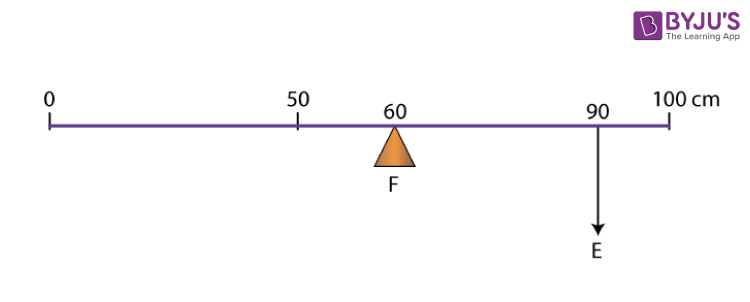(a) State with reasons whether the weight W of the rule is greater than, less than or equal to the effort E.

(b) Find the mechanical advantage in an ideal case.

Solutions:

(a) The weight W of the scale is greater than E

The arm on the side of effort E is 30 cm and on the side of the weight of scale is 10 cm. So, in order to balance the scale, weight W of scale should be more than effort E

(b) M.A. = Effort arm / Load arm

Effort arm = 30 cm

Thus M.A. = 30 / 10

= 3

Question: 24

Which type of lever has a mechanical advantage always more than 1? Give reason with one example. What change can be made in this lever to increase its mechanical advantage?

Solutions:

The mechanical advantage of Class II levers are always more than 1

Example: a paper cutter

We can increase the length of effort arm in this lever to increase its mechanical advantage.

Question: 25

Draw a diagram of a lever which is always used as a force multiplier. How is the effort arm related to the load arm in such a lever?

Solutions: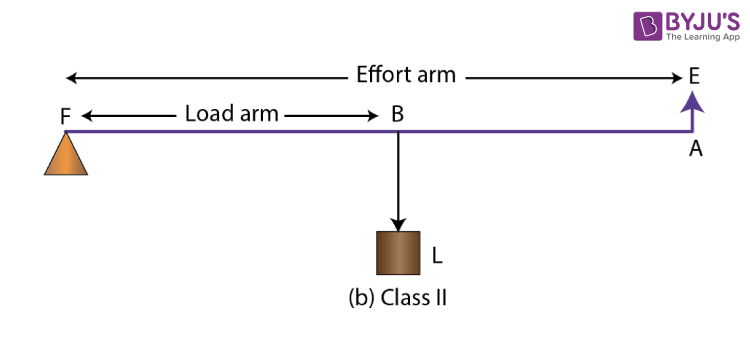In this type of lever, the effort arm is longer than the load arm

Question: 26

Explain why the mechanical advantage of a class II type of lever is always more than 1.

Solutions:

In a Class II type of levers, the load L is in between the effort E and the fulcrum F. So, the effort arm is always longer than the load arm. Hence, M.A. > 1

Questions: 27

Draw a labelled diagram of a Class II lever. Give one example of such a lever.

Solutions: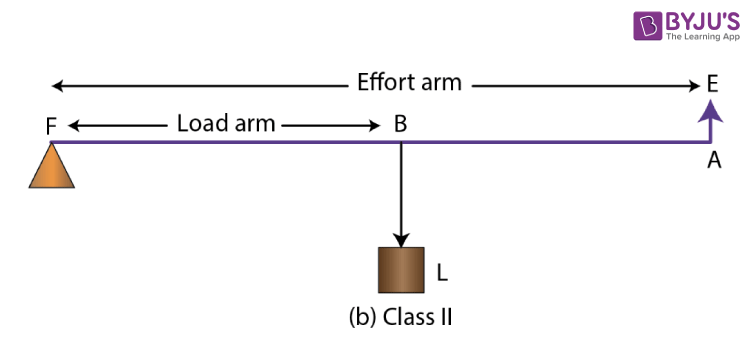Example: a wheelbarrow

Question: 28

Shows a lemon crusher.

(a) In the diagram, mark the position of the directions of load L and effort E.

(b) Name the class of lever.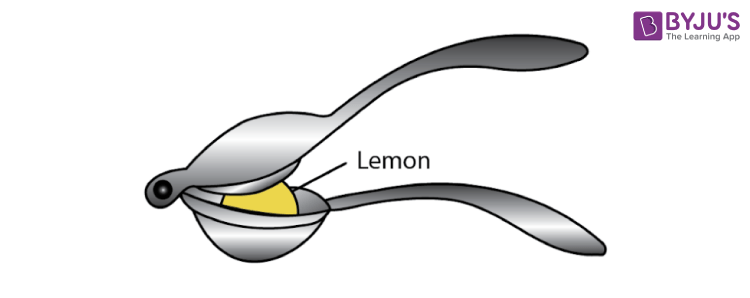Solutions:

(a)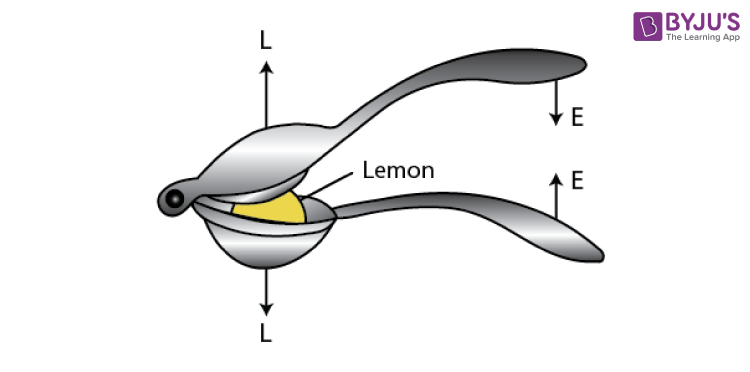(b) It is a class II lever.

Question: 29

The diagram below shows a rod lifting a stone.

(a) Mark position of fulcrum F and draw arrows to show the directions of load L and effort E.

(b) What class of lever is the rod?

(c) Give one more example of the same class of lever stated in part (b).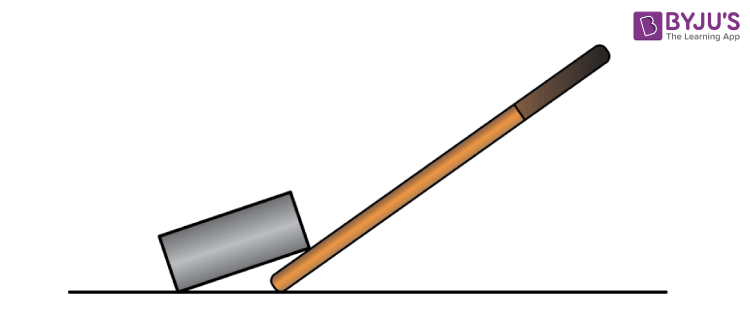Solutions: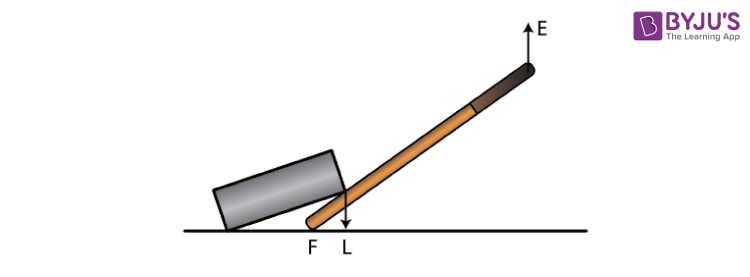(b) Here the load is between fulcrum and effort. So, this rod is a class II lever.

(c) A wheelbarrow is an example of a class II lever.

Question: 30

State the kind of lever which always has the mechanical advantage less than 1. Draw a labelled diagram of such a lever.

Solutions:

The mechanical advantage of Class III levers are always less than 1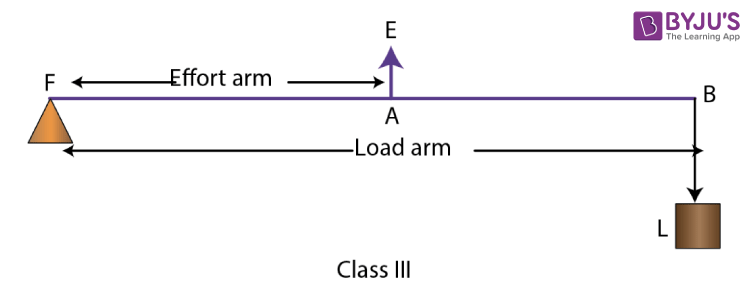Question: 31

Explain why the mechanical advantage of the class III lever is always less than 1.

Solutions:

In Class III lever, the effort E is in between the fulcrum F and the load L. So, the effort arm is always smaller than the load arm. Hence, M.A. < 1

Question: 32

Classes III levers have mechanical advantage less than 1. Why are they then used?

Solutions:

With levers of Class III, we do not get gain in force, but we get gain in speed, i.e a larger displacement of load is obtained by a smaller displacement of effort.

Question: 33

Draw a labelled sketch of a class III lever. Give one example of this kind of lever.

Solutions:Example: Knife

Question: 34

State the class of levers and the relative positions of load (L), effort (E) and fulcrum (F) in

(a) a bottle opener, and

(b) sugar tongs.

Solutions:

(a) A bottle opener is a lever of Class II, as the fulcrum F and the effort E are at the two ends of the lever and the load is in between the fulcrum F and the effort E.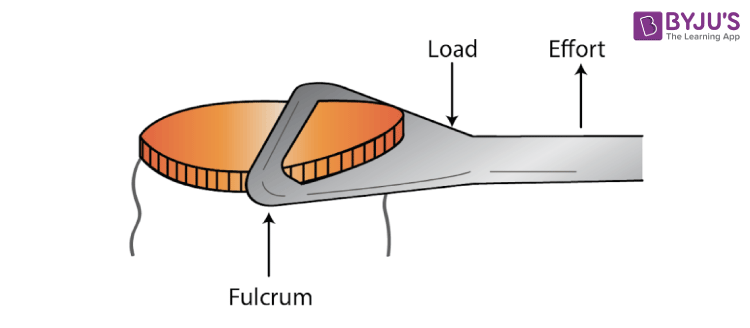Bottle opener

(b) Sugar tongs is a lever of Class III, as the fulcrum F and the load L are at the two ends of the lever and the effort E is in between the fulcrum F and the load L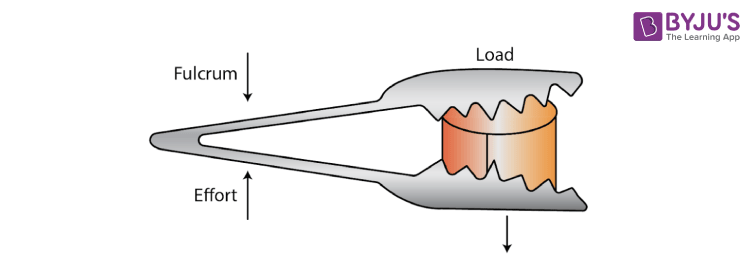Sugar tongs

Question 35:

Draw diagrams to illustrate the position of fulcrum, load and effort, in each of the following:

(a)A seesaw

(b)A common balance

(c)A nutcracker

(d)Forceps.

Solutions:

(a) A seesaw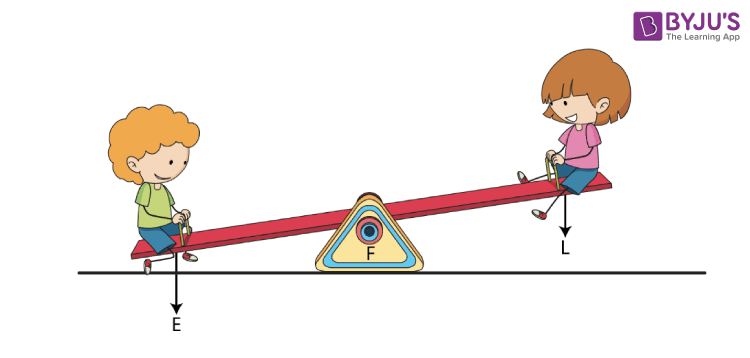(b) A common balance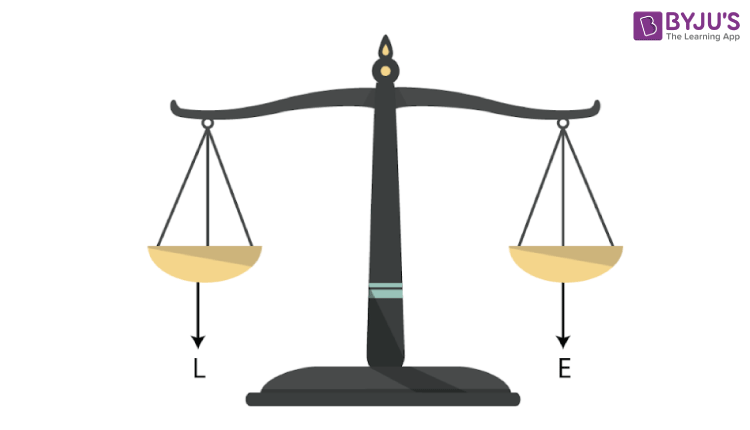(c) A nutcracker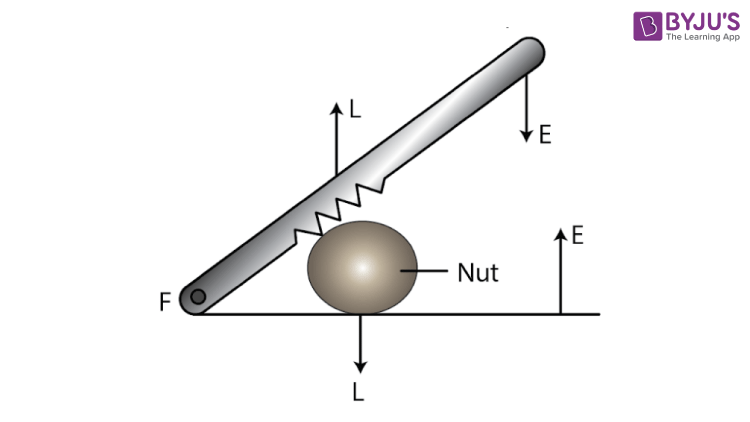(d) Forceps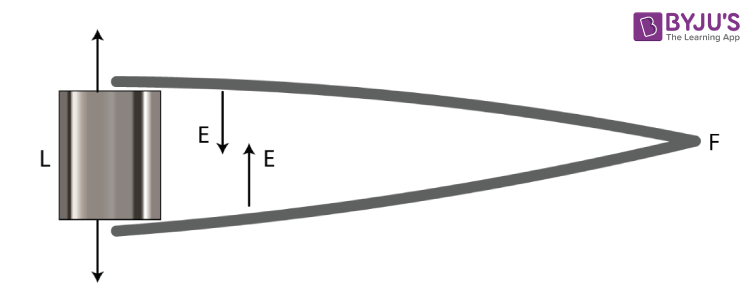Question: 36

Classify the following into levers as class I, class II or class III:

(a) A door

(b) A catapult

(c) A wheelbarrow

(d) A fishing rod.

Solutions:

(a) A door – Class II

(b) A catapult – Class I

(c) A wheelbarrow – Class II

(d) A fishing rod – Class III

Question: 37

What type of lever is formed by the human body while (a) raising a load on the palm, and (b) raising the weight of body on toes?

Solutions:

(a) Raising a load on the palm is a Class III lever

Here, the elbow of the human arm is the fulcrum. Biceps exert the effort in the middle and load on the palm is at the other end.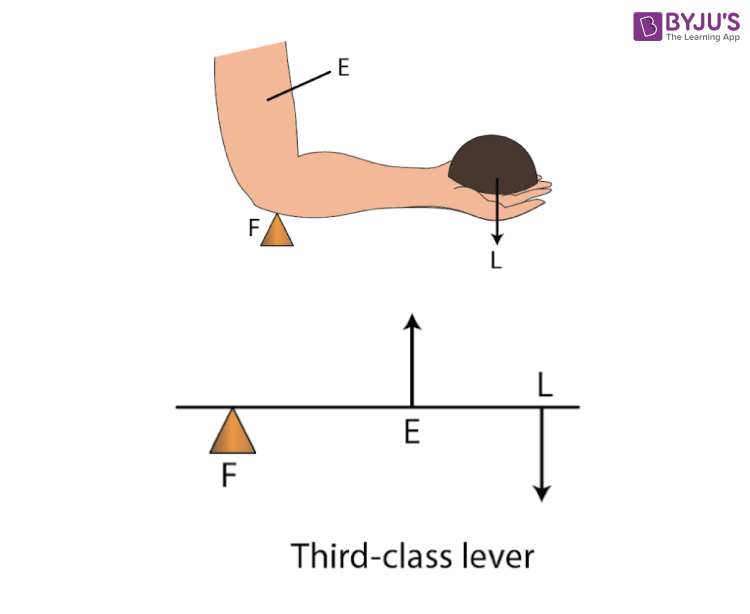(b) Raising a weight of body on toes is a Class II lever

The fulcrum is at toes at one end, the load that is the weight of the body is in the middle and the effort by muscles is at the other end.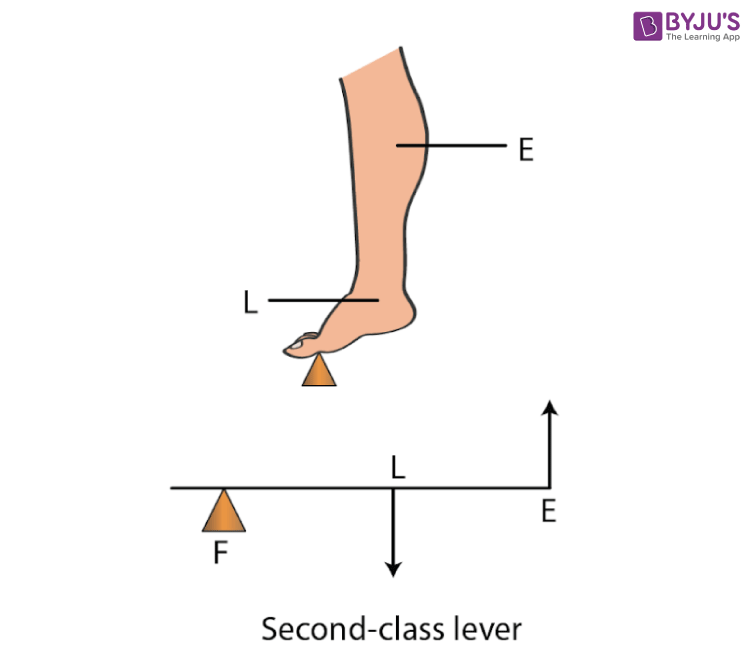Question: 38

Indicate the positions of load L, effort E and fulcrum F in the forearm shown below. Name the class of lever.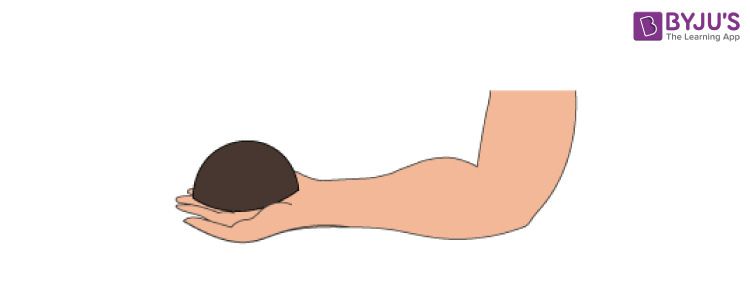Solutions: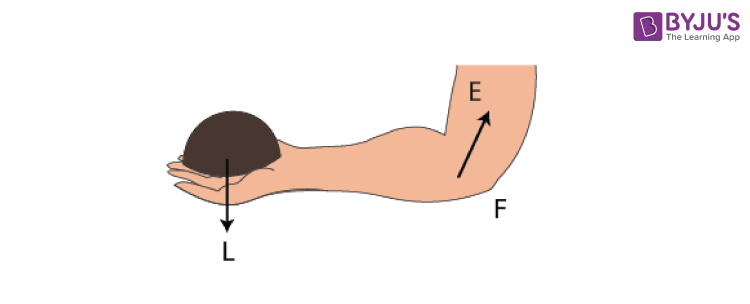It is a Class III lever

Question: 39

Give an example of each class of lever in a human body.

Solutions:

The examples of each class of lever in a human body are as follows

(i) The action of nodding of the head is a Class I lever. Here the spine acts as the fulcrum, load is at its front part and effort is at its rear part.

(ii) Raising the weight of the body on toes is a Class II lever. Here the fulcrum is at toes at one end, the load is in the middle and effort by muscles is at the other end.

(iii) Raising a load by forearm is a Class III lever. Here the elbow joint acts as fulcrum at one end, biceps exerts the effort in the middle and a load on the palm is at the other end.

Question: 40

Complete the following sentences:

(a) Mechanical advantage = ___________ × velocity ratio

(b) In class II lever, effort arm is __________ than the load arm.

(c) A scissors is a ___________ multiplier.

Solutions:

(a) Mechanical advantage = efficiency × velocity ratio

(b) In Class II lever, effort arm is longer than the load arm.

(c) A scissors is speed multiplier

MULTIPLE CHOICE TYPE

Question: 1

a. M.A. = L x E

b. M.A. x E = L

c. E = M.A. x L

d. None of these

Solutions:

M.A × E = L

Question: 2

The correct relationship between the mechanical advantage (M.A.), velocity ratio (V.R.) and efficiency (η) is:

a. M.A. = η x V.R.

b. V.R. = η x M.A.

c. η = M.A. x V.R.

d. None of these

Solutions:

The correct relationship between the mechanical advantage (M.A), velocity ratio (V.R) and efficiency (η) is

M.A. = η × V.R.

Question: 3

Select the incorrect statement:

(a) A machine always has the efficiency less than 100%.

(b) The mechanical advantage of a machine can be less than 1.

(c) A machine can be used as a speed multiplier.

(d) A machine can have a mechanical advantage greater than the velocity ratio.

Solutions:

The incorrect statement is-

A machine can have a mechanical advantage greater than the velocity ratio.

Question: 4

The lever for which the mechanical advantage is less than 1 has the:

(a) Fulcrum at mid-point between load and effort.

(b) Load between effort and fulcrum.

(c) Effort between fulcrum and load.

(d) Load and effort acting at the same point.

Solutions:

The lever for which the mechanical advantage is less than 1 has the effort between fulcrum and load

Question: 5

Class II levers are designed to have:

a. M.A. = V.R.

b. M.A. > V.R.

c. M.A. > 1

d. M.A. < 1

Solutions:

Class II levers are designed to have-

M.A. > 1

NUMERICAL

Question: 1

A crowbar of length 120 cm has its fulcrum situated at a distance of 20 cm from the load. Calculate the mechanical advantage of the crowbar.

Solutions:

Given

Total length of a crowbar = 120 cm

Effort arm = 120 – 20

= 100 cm

M.A. = 100 / 20

= 5

Question: 2

A pair of scissors has its blades 15 cm long, while its handles are 7.5 cm long. What is its mechanical advantage?

Solutions:

Given

Effort arm = 7.5 cm

= 7.5 / 15

= 0.5

Question: 3

A force of 5kgf is required to cut a metal sheet. A shears used for cutting the metal sheet has its blades 5 cm long, while its handle is 10 cm long. What effort is needed to cut the sheet?

Solutions:

Given

Effort arm = 10 cm

= 10 / 5

= 2

To find effort, we have

= 5 / 2

= 2.5 kgf

Question: 4

The diagram below shows a lever in use.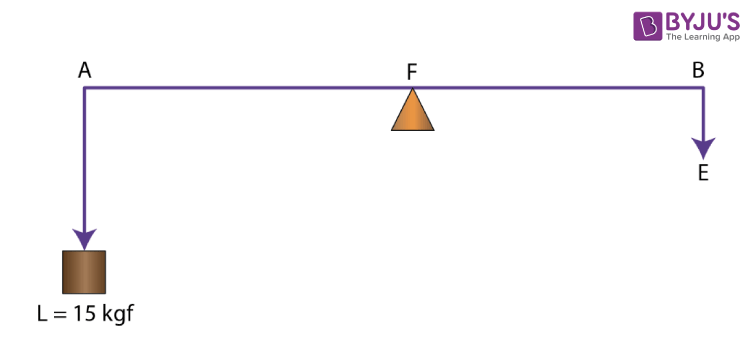(a) To which class of lever does it belong?

(b) If AB =1 m, AF= 0.4 m, find its mechanical advantage.

(c) Calculate the value of E.

Solutions:

(a) This belongs to a Class I lever

(b) Given AB = 1m

AF = 0.4 m

BF = 0.6 m

Mechanical advantage M.A = BF / AF

= 0.6 / 0.4

= 1.5

(c) Given Load = 15 kgf

= 15 / 1.5

= 10 kgf

Question: 5

A man uses a crowbar of length 1.5 m to raise a load of 75kgf by putting a sharp edge below the bar at a distance 1 m from his hand. (a) Draw a diagram of the arrangement showing the fulcrum (F), load (L) and effort (E) with their directions. (b) State the kind of lever. (c) Calculate: (i) load arm, (ii) effort arm, (iii) mechanical advantage, and (iv) the effort needed.

Solutions: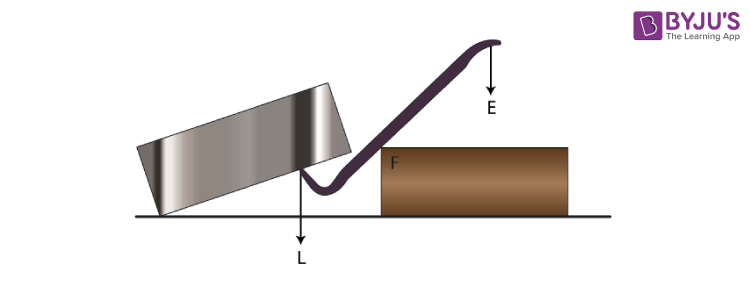Crowbar is a class I lever

Given

(i) Total length of crowbar = 1.5 m

Effort arm = 1 m

Load arm = 1.5 – 1

= 0.5 m

(ii) Effort arm = 1 m

= 1 / 0.5

= 2

(iv) The effort needed

= 75 / 2

= 37.5 kgf

Question: 6

A pair of scissors is used to cut a piece of a cloth by keeping it at a distance 8.0 cm from its rivet and applying an effort of 10 kgf by fingers at a distance 2.0 cm from the rivet.

(a) Find: (i) the mechanical advantage of scissors and (ii) the load offered by the cloth.

(b) How does the pair of scissors act: as a force multiplier or as a speed multiplier?

Solutions:

Effort arm = 2 cm

Given effort = 10 kgf

= 2 / 8

= 0.25

(ii) Load = M.A. × effort

= 0.25 × 10

= 2.5 kgf

The pair of scissors acts as a speed multiplier since the mechanical advantage is less than 1 i.e M.A < 1

Question: 7

A 4 m long rod of negligible weight is to be balanced about a point 125 cm from one end and a load of 18 kgf is suspended at a point 60 cm from the support on the shorter arm.

(a) If a weight W is placed at a distance of 250 cm from the support on the long arm, Find W.
(b) If a weight 5 kgf is kept to balance the rod, find its position.
(c) To which class of lever does it belong?

Solutions:

Given

Total length of rod = 4 m = 400 cm

(a) 18 kgf load is placed at 60 cm from the support.

W kgf weight is placed at 250 cm from the support.

According to the principle of moments

18 × 60 = W × 250

W = 4.32 kgf

(b) Given W = 5 kgf

18 kgf weight is placed at 60 cm from the support.

Let 5 kg of weight is placed at d cm from the support.

According to the principle of moments

18 × 60 = 5 × d

d = 216 cm from the support on the long arm

(c) It belongs to class I lever

Question: 8

A lever of length 9 cm has its load arm 5 cm long and the effort arm is 9 cm long. (a) To which class does it belong? (b) Draw diagram of the lever showing the position of fulcrum F and directions of both the load L and effort E. (c) What is the mechanical advantage and velocity ratio if the efficiency is 100%? (d) What will be the mechanical advantage and velocity ratio if the efficiency becomes 50%?

Solutions:

(a) Length of the lever is equal to the effort arm. The effort arm is also more than the load arm. Hence this is a class II lever.

(b)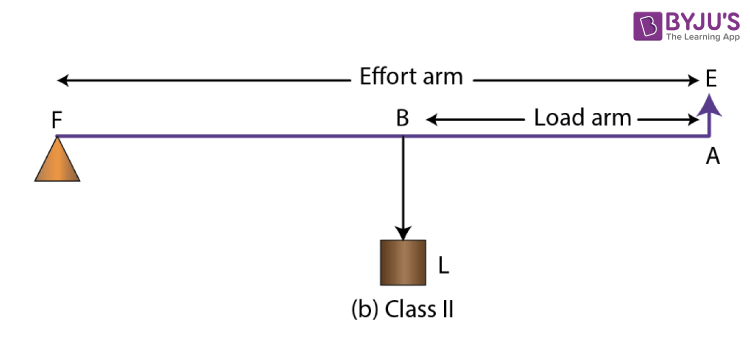M.A. = Effort arm / Load arm

= 9 cm / 5 cm

M.A. = 1.8

Relation between mechanical advantage, efficiency and velocity ratio is

M.A. = η × V.R.

M.A.= V.R. (η = 100% = 1)

(d) When efficiency reduces, its mechanical advantage reduces and velocity ratio remains the same. So, when efficiency becomes 50%, M.A. = 0.9 and V.R. = 1.8

Question: 9

The diagram below shows a lever in use.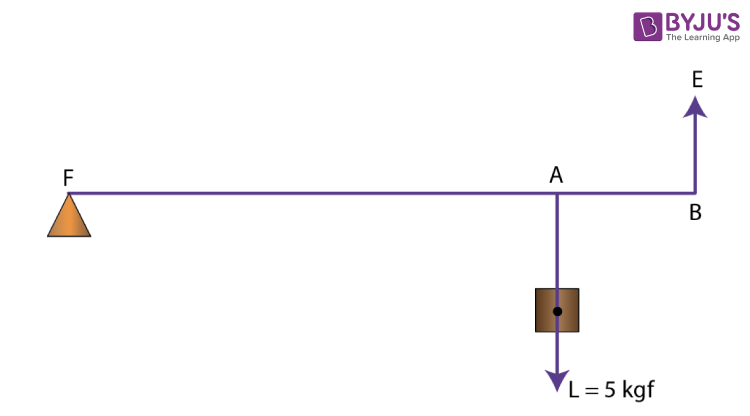(a) To which class of lever does it belong?
(b) If FA = 80 cm, AB = 20 cm, find its mechanical advantage.
(c) Calculate the value of E.

Solutions:

(a) It belongs to class II lever.

(b) Given FA = 80 cm

AB = 20 cm

BF = FA + AB

= 100 cm

Mechanical advantage M.A. = BF / AF

= 100 / 80

= 1.25

(c) Effort (E) = Load (L) / M.A.

= 5 / 1.25

= 4 kgf

Question: 10

The figure shows a wheelbarrow of mass 15 kg carrying a load of 30 kgf with its centre of gravity at A. The points B and C are the centre of wheel and tip of the handle such that the horizontal distance AB = 20 cm and AC = 40 cm.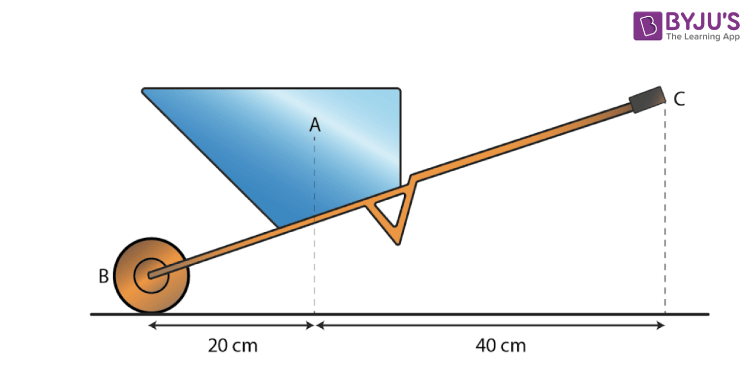Find: (a) the load arm, (b) the effort arm, (c) the mechanical advantage, and (d) the

minimum effort required to keep the leg just off the ground.

Solutions:

(a)

(i) Load arm AF = 20 cm

(ii) Effort arm CF = 60 cm

(iii) Mechanical advantage M.A. = CF / AF

= 60 / 20

= 3

(iv) Total load = 30 + 15

= 45 kgf

= (30 + 15) / 3

= 15 kgf

Question: 11

The diagram below shows the use of a lever.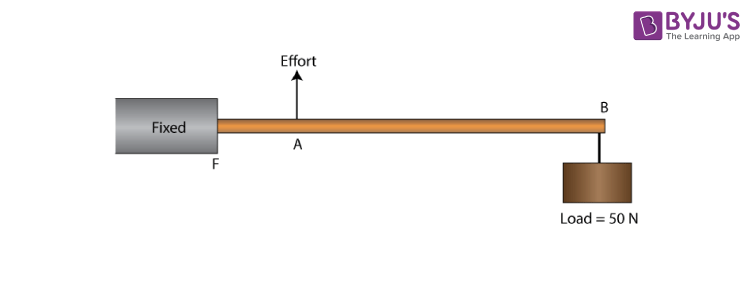(a) State the principle of moments as applied to the above lever.

(b) To which class of lever does it belong? Give an example of this class of lever.

(c) If FA = 10cm, AB = 490cm, calculate: (i) the mechanical advantage, and (ii) the minimum effort required to lift the load (= 50N).

Solutions:

(a) According to the principle of moments

load × FB = Effort × FA

(b) It belongs to Class III lever. The example of this lever is a knife.

(c) Given FA = 10 cm

AB = 490 cm

BF = 490 + 10

= 500 cm

M.A= AF / BF

= 10 / 500

= 1 / 50

The minimum effort required to lift the load

= 50 / 1 / 50

= 50 × 50

= 2500 N

Question: 12

A fire tongs has its arms 20 cm long. It is used to lift a coal of weight 1.5kgf by applying an effort at a distance 15 cm from the fulcrum. Find: (i) the mechanical advantage of fire tongs and (ii) the effort needed.

Solutions:

Given

Fire tongs has its arms = 20 cm

Effort arm = 15 cm

= 15 / 20

= 0.75

(ii) Effort = Load / M.A

= 1.5 / 0.75

= 2.0 kgf

EXERCISE 3(b) PAGE NO: 66

Question: 1

What is a fixed pulley? State its one use.

Solutions:

Fixed pulley is defined as ‘a pulley which has its axis of rotation stationary in position is called a fixed pulley. This type of pulley is used lifting a small load such as a water bucket.

Question: 2

What is the ideal mechanical advantage of a single fixed pulley? Can it be used as a force multiplier?

Solutions:

The mechanical advantage of a single fixed pulley is 1. No, it cannot be used as a force multiplier.

Question: 3

Name the pulley which has no gain in mechanical advantage. Explain, why is such a pulley is then used?

Solutions:

In single fixed pulley, there is no gain in mechanical advantage. A single fixed pulley is used only to change the direction of effort to be applied i.e with its use the effort can be applied in a more convenient direction. It is difficult to lift a load up directly upwards.

Question: 4

What is the velocity ratio of a single fixed pulley?

Solutions:

The velocity ratio of a single fixed pulley is 1

Question: 5

In a single fixed pulley, if the effort moves by a distance x downwards, by what height is the load raised upwards?

Solutions:

The load rises with the distance x upwards.

Question: 6

What is a single movable pulley? What is its mechanical advantage in the ideal case?

Solutions:

A single pulley whose axis of rotation is movable is called a single movable pulley. The mechanical advantage in the ideal case is 2

Question: 7

Name the type of single pulley that has an ideal mechanical advantage equal to 2. Draw a labeled diagram of the pulley mentioned by you.

Solutions:

The single movable pulley acts as a force multiplier.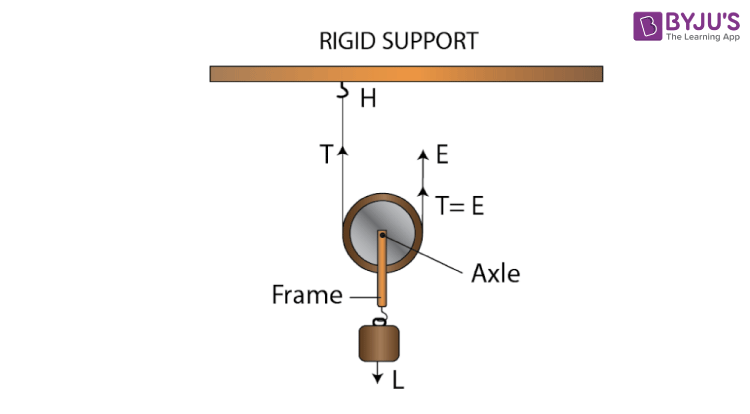Question: 8

Give two reasons why the efficiency of a single movable pulley system is not 100%.

Solutions:

The efficiency of a single movable pulley system is not 100% because of the following reasons

(i) The friction of the pulley bearing is not zero

(ii) The weight of the pulley and string is not zero

Question: 9

In which direction the force need be applied, when a single pulley is used with a mechanical advantage greater than 1? How can you change the direction of force applied without altering its mechanical advantage? Draw a labelled diagram of the system.

Solutions:

The force needs to be applied in an upward direction.

By using a single movable pulley along with a fixed pulley the direction of the applied force can be changed without altering its mechanical advantage.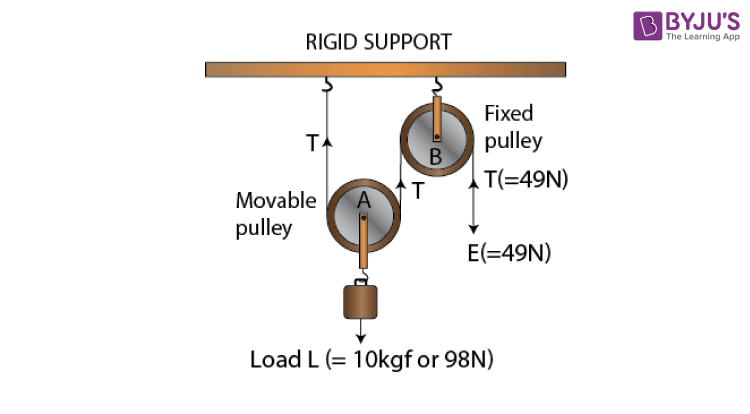Question: 10

What is the velocity ratio of a single movable pulley? How does the friction in the pulley bearing affect it?

Solutions:

The velocity ratio of a single movable pulley is always 2. The friction in the pulley bearing has no effect.

Question: 11

In a single movable pulley, if the effort moves by a distance x upwards, by what height is the load raised?

Solutions:

The load is raised to a height of x / 2

Question: 12

Draw a labelled diagram of an arrangement of two pulleys, one fixed and other moveable. In the diagram, mark the directions of all forces acting on it. What is the ideal mechanical advantage of the system? How can it be achieved?

Solutions: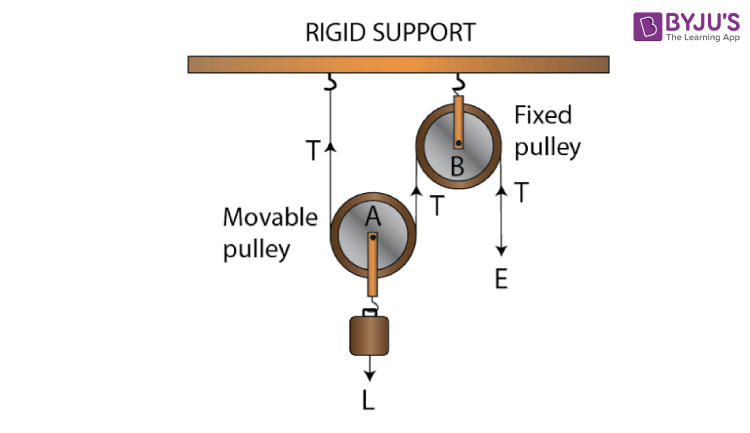The ideal mechanical advantage of this system is 2. This can be achieved by assuming that string and the pulley are massless and also there is no friction in the pulley bearings between the string and surface of the rim of the pulley.

Question: 13

The diagram alongside shows a pulley arrangement.

(a) Name the pulleys A and B.
(b) In the diagram, mark the direction of tension on each strand of string.
(c) What is the purpose of the pulley B?
(d) If the tension is T, deduce the relation between (i) T and E, and (ii) E and L.
(e) What is the velocity ratio of the arrangement?
(f) Assuming that the efficiency of the system is 100%, what is the mechanical advantage?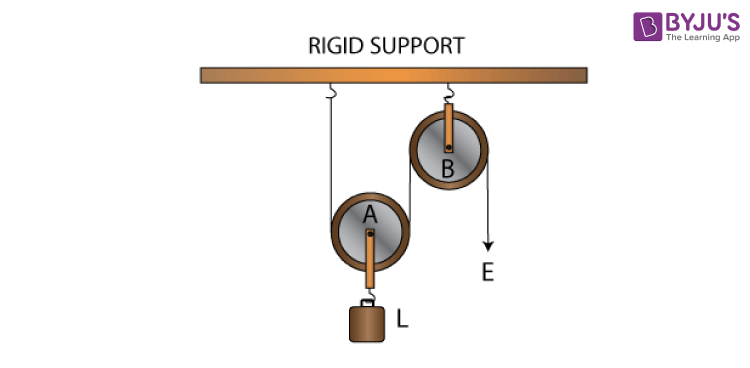Solutions:

(a)(b) The purpose of fixed pulley B is to change the direction of effort to be applied from upward to downward

(c) At the free end, the effort E balances the tension T. So, E = T

(d) The velocity ratio of this arrangement is 2

(e) If the efficiency of the system is 100%, the mechanical advantage is 2

Question: 14

State four differences between a single fixed pulley and a single movable pulley.

Solutions:

 Single fixed pulley Single movable pulley It is fixed to a rigid support It is not fixed to a rigid support Its ideal mechanical advantage is 1 Its ideal mechanical advantage is 2 Its velocity ratio is 1 Its velocity ratio is 2 The weight of pulley itself does not affect its mechanical advantage The weight of pulley itself reduces its mechanical advantage It is used to change the direction of effort from upwards to downwards It is used as a force multiplier

Question: 15

The diagram alongside shows and arrangement of three pulleys A, B and C. The load is marked as L and the effort as E.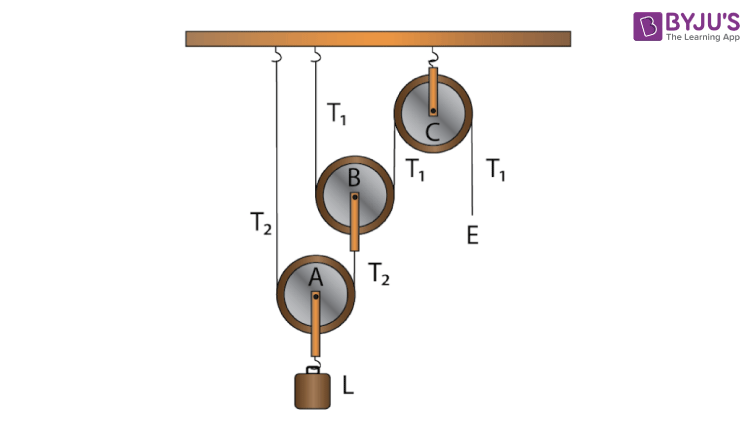(a) Name the pulleys A, B and C.

(b) Mark in the diagram the direction of load (L), effort (E) and tension T1 and T2 in the two strings.

(c) How are the magnitudes of L and E related to the tension T1?

(d) Calculate the mechanical advantage and velocity ratio of the arrangement.

(e) What assumptions have you made in parts (c) and (d)?

Solutions:

(a) Pulleys A and B are movable pulleys while pulley C is a fixed pulley.

(b)(c) The magnitude of effort E = T1 and the magnitude of L = 22 T1 = 4T1

(d) The mechanical advantage = 22 = 4 and the velocity ratio = 22 = 4

(e) The pulleys A and B are weightless and there is no friction

Question: 16

Draw a diagram of combination of three movable pulleys and one fixed pulley to lift up a load. in the diagram, show the directions of load, effort and tension in each strand. Find: (i) the mechanical advantage, (ii) velocity ratio and (iii) the efficiency of the combination in ideal situation.

Solutions: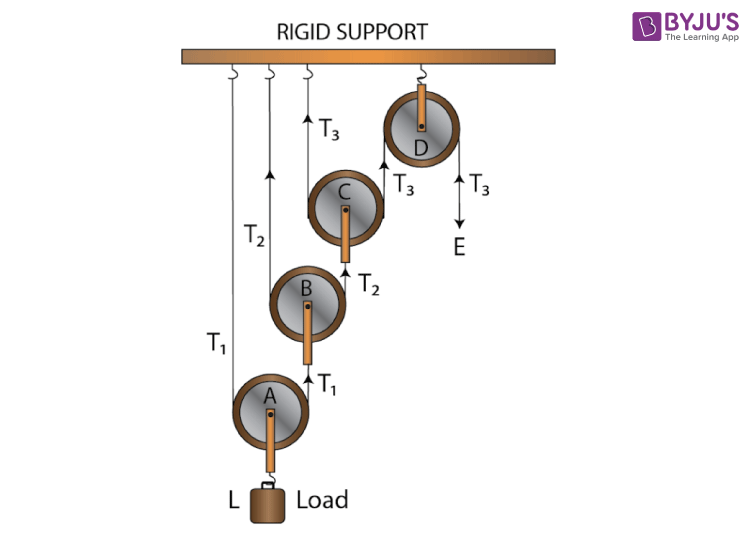Tension T1 in the string passing over the pulley A is given as

2T1 = L

T1 = L / 2

Tension T2 in the string passing over the pulley B is given as

2T2 = T1

T2= T1 / 2

T2 = L / 22

Tension T3 in the string passing over the pulley C is given as

2T3 = T2

T3 = T2 / 2

T3 = L / 23

In equilibrium, T3 = E

E = L / 23

Mechanical advantage M.A = L / E = 23

As one end of each string passing over a movable pulley is fixed, so the other end of string moves up twice the distance moved by the axle of the movable pulley.

If the load L moves up by a distance x i.e dL = x and

The effort moves by a distance 23x or dE = 23x

Velocity Ratio VR = (Distance moved by the effort dE) / (Distance moved by the load dL)

= 23x / x

= 23

Efficiency = M.A / V.R

= 23 / 23

= 1 or 100%

Question: 17

Draw a diagram of a block and tackle system of pulleys having a velocity ratio of 5. In your diagram indicate clearly the points of application and the directions of the load L and effort E. Also mark the tension T in each strand.

Solutions: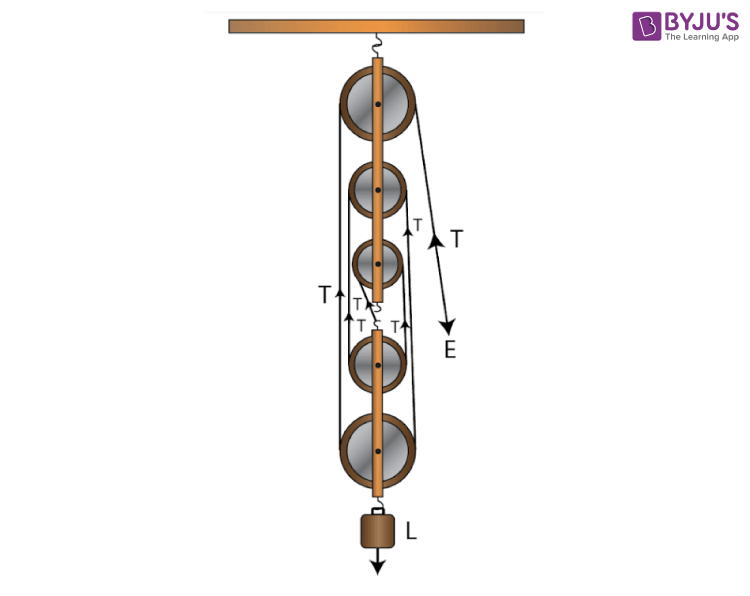Question: 18

Give reasons for the following:

(a) In a single fixed pulley, the velocity ratio is always more than the mechanical advantage.
(b) The efficiency of a movable pulley is always less than 100%.
(c) In the case of a block and tackle system, the mechanical advantage increases with the increase in the number of pulleys.
(d) The lower block of a block and tackle pulley system must be of negligible weight.

Solutions:

(a) Some effort is wasted in a single fixed pulley in overcoming friction between the strings and the grooves of the pulley. So, the effort needed is greater than the load and thus the velocity ratio is always more than the mechanical advantage

(b) The efficiency of a movable pulley is always less than 100% because some effort is wasted in overcoming the friction between the strings and the grooves of the pulley.

(c) The mechanical advantage increases with the increase in the number of pulleys because the mechanical advantage is equal to the total number of pulleys in both the blocks.

(d) The efficiency depends upon the mass of the lower block, hence efficiency is reduced due to the weight of the lower block of pulleys.

Question: 19

Name a machine which is used to:

(a)Multiply force

(b)Multiply speed, and

(c)Change the direction of force applied.

Solutions:

(a) Multiply force – a movable pulley is used

(b) Multiply speed – Class III lever

(c) Change the direction of force applied – single fixed pulley

Question: 20

State whether the following statements are true or false.

(a) The velocity ratio of a single fixed pulley is always more than 1.

(b) The velocity ratio of a single movable pulley is always 2.

(c) The velocity ratio of a combination of n movable pulleys with a fixed pulley is always 2n.

(d) The velocity ratio of a block and tackle system is always equal to the number of strands of the tackle supporting the load.

Solutions:

(a) The velocity ratio of a single fixed pulley is always more than 1 (False)

(b) The velocity ratio of a single movable pulley is always 2 (True)

(c) The velocity ratio of a combination of n movable pulleys with a fixed pulley is always 2n (True)

(d) The velocity ratio of a block and tackle system is always equal to the number of strands of the tackle supporting the load (True)

MULTIPLE CHOICE TYPE

Question: 1

A single fixed pulley is used because it:

(a) Has a mechanical advantage greater than 1
(b) Has a velocity ratio less than 1
(c) Gives 100% efficiency
(d) Helps to apply the effort in a convenient direction.

Solutions:

A single fixed pulley is used because it (d) helps to apply the effort in a convenient direction.

Question: 2

The mechanical advantage of an ideal single movable pulley is:

(a) 1
(b) 2
(c) less than 2
(d) less than 1.

Solutions:

The mechanical advantage of an ideal single movable pulley is 2

Derivation: Consider the diagram given below: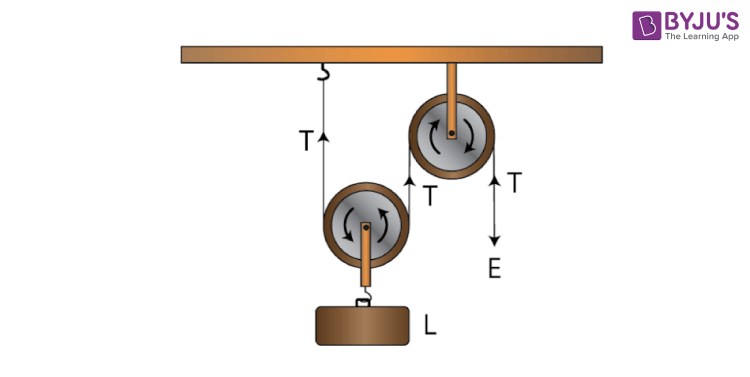In the figure above, the load L is balanced by the tension T in two segments of the string and the effort E balances the tension T at the free end, so

L + L = 2T

E = T

Assumption: Weight of the pulley is negligible

M.A. = Load L / Effort E

= 2T / T

= 2

Hence, a single movable pulley has an M.A equal to 2

Question: 3

A movable pulley is used as :

(a) a force multiplier
(b) a speed multiplier
(c) a device to change the direction of effort
(d) an energy multiplier

Solutions:

A movable pulley is used as a force multiplier

Explanation: The mechanical advantage of the movable pulley is greater than 1. Hence, using a single movable pulley, the load can be lifted by applying an effort equal to half the load i.e the single movable pulley acts as a force multiplier.

NUMERICALS

Question: 1

A woman draws water from a well using a fixed pulley. The mass of the bucket and water together is 6 kg. The force applied by the women is 70 N. calculate the mechanical advantage. (Take g = 10 m s-2).

Solutions:

Given

The force applied by the woman is = 70 N

The mass of bucket and water together is = 6 kg

Total load = 6 × 10

= 60 N

= 60 / 70

= 0.857

Question: 2

A fixed pulley is driven by a 100 kg mass falling at a rate of 8.0 m in 4.0 s. It lifts a load of 75.0 kgf. Calculate:

(a) The power input to the pulley taking the force of gravity on 1 kg as 10 N.

(b) the efficiency of the pulley, and

(c) the height to which the load is raised in 4.0 s.

Solutions:

(a) Effort driving the pulley is,

E = mg

= 100 × 10

= 1000 N

Input power is,

Pin = 2000 W

(b) Load pulley by the pulley is,

L = 75 × 10

= 750 N

Hence, M.A is,

M.A = L / E

= 750 / 1000

= 0.75

When the effort moves by a distance d downwards, the load moves by the same distance upwards. Thus,

V.R = 1

Therefore efficiency is,

η = M.A. / V.R.

= 0.75 / 1

= 0.75

= 75%

(c) The load moves by the same distance upwards when the effort moves by a distance d downwards. So, height to which the load moves is 8 m

Question: 3

A single fixed pulley and a movable pulley both are separately used to lift a load of 50 kgf to the same height. Compare the efforts applied.

Solutions:

In case of a single fixed pulley, the effort i.e Ef needed to lift a load is equal to the load itself.

Hence, Ef = L

Ef = L

The effort needed to lift a load is equal to half the load

Em = 50 kgf / 2

= 25 kgf

Hence, the ratio of efforts applied by the respective pulley is

Ef / Em = 2 / 1

Therefore Ef : Em = 2: 1

Question: 4

In a block and tackle system consisting of 3 pulleys, a load of 75 kgf is raised with an effort of 25 kgf. Find: (i) the mechanical advantage, (ii) the velocity ratio, and (iii) the efficiency.

Solutions:

Given,

Effort = 25 kgf

n = 3

= 75 / 25

= 3

M.A. = n = 3

Velocity V.R. = n = 3

Efficiency η = M.A. / V.R.

= 3 / 3

= 1 or 100%

Question: 5

A block and tackle system has 5 pulleys. If an effort of 1000 N is needed in the downward direction to raise a load of 4500 N, calculate:

(b)The velocity ratio and,

(c)The efficiency of the system.

Solutions:

Given,

A block and tackle system has 5 pulleys (n = 5)

Effort = 1000 N

= 4500 / 1000

= 4.5

(b) The velocity ratio = n = 5

(c) The efficiency of the system η = M.A. / V.R

= 4.5 / 5

= 0.9

= 90%

Question: 6

In figure draw a tackle to lift the load by applying the force in the download direction.

(a) Mark in the diagram the direction of load L and effort E.

(b) If the load is raised by 1 m, through what distance will the effort move?

(c) State how many strands of tackle are supporting the load.

(d) What is the mechanical advantage of the system?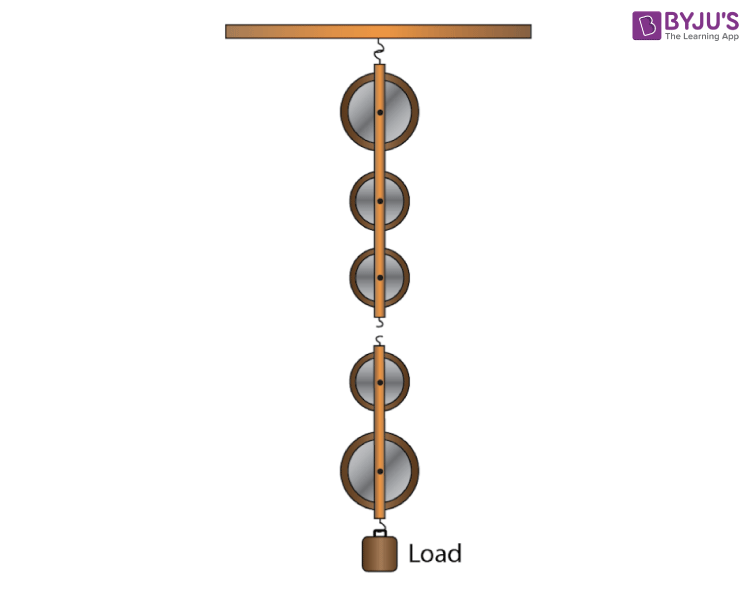Solutions:

(a)(b) If the load is raised by 1 m, the effort move = 1 × 5 = 5 m

(c) Five strands of tackle are supporting the load

(d) The mechanical advantage of the system is given by

= 5T / T

= 5

Question: 7

A pulley system has a velocity ratio 3. Draw a diagram showing the point of application and direction of load (L), effort (E) and tension (T). If lifts a load of 150 N by an effort of 60 N. Calculate its mechanical advantage. Is the pulley system ideal? Give reason.

Solutions: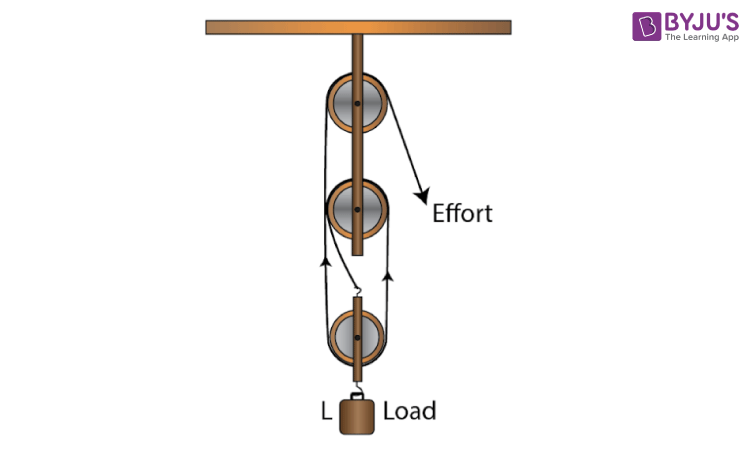Effort = 60 N

= 150 / 60

= 2.5

The pulley system is not ideal because the mechanical advantage is less than 3

Question: 8

Figure shows a system of four pulleys. The upper two pulleys are fixed and the lower two are movable.

(a) Draw a string around the pulleys. Also show the point of application and direction in which the effort E is applied.
(b) What is the velocity ratio of the system?
(c) How are load and effort of the pulley system related?
(d) What assumption do you make in arriving at your answer in part (c)?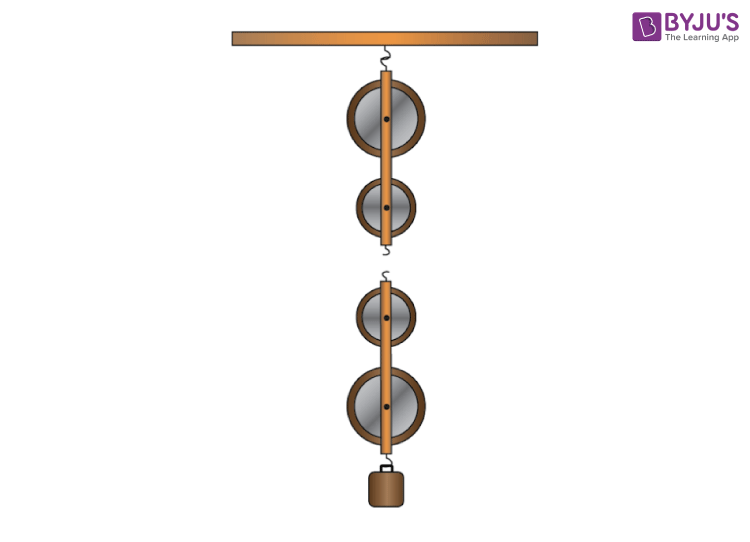Solutions:

(a)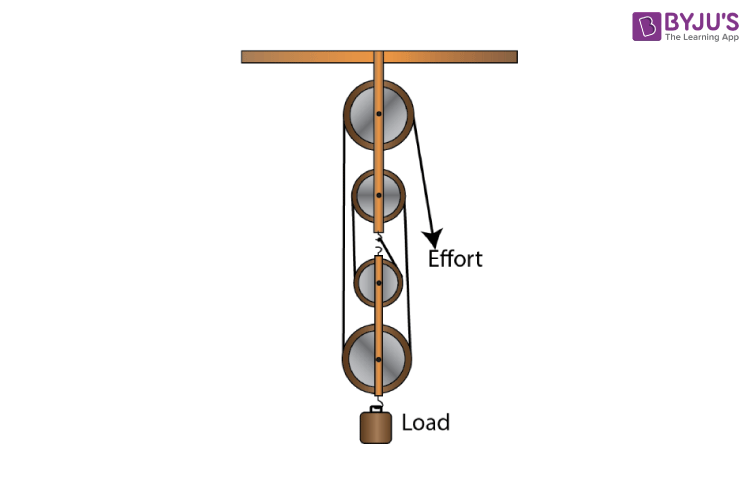(b) Velocity ratio of the system = n = 4

(c) The relation between load and effort

M.A. = load / effort = n = 4

(d)

(i) There is no friction in the pulley bearings

(ii) Weight of lower pulleys is negligible and

(iii) The effort is applied downwards.

Question: 9

Figure shows a block and tackle system of pulleys used to lift a load.

(a) How many strands of tackle are supporting the load?
(b) Draw arrows to represent tension T in each strand.
(c) What is the mechanical advantage of the system?
(d) When load is pulled up by a distance 1 m, how far does the effort end move?
(e) How much effort is needed to lift a load of 100 N?Solutions:

(a) There are 4 strands of tackle supporting the load.

(b)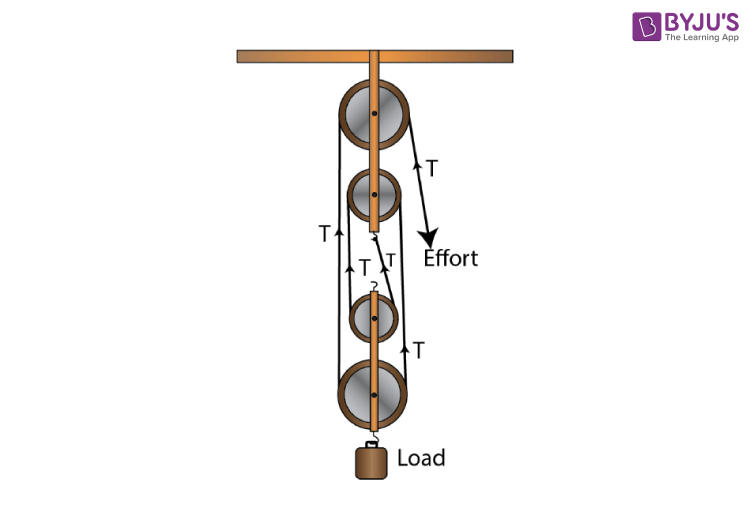(c) The mechanical advantage of the system

= 4T / T

= 4

(d) When load is pulled up by a distance 1 m, the effort end will move by a distance

= 1 × 4 = 4m

(e) M.A = Load / Effort

4 = 100 / effort

Effort = 100 / 4

= 25 N

Question: 10

A block and tackle system has the velocity ratio 3. Draw a labelled diagram of the system indicating the points of application and the directions of load L and effort E. A man can exert a pull of 200 kgf. (a) What is the maximum load he can raise with this pulley system if its efficiency is 60%? (b) If the effort end moves a distance 60 cm, what distance does the load move?

Solutions:

Given,

A block and tackle system has V.R = n (number of pulleys)

Hence, n = 3

The diagram is shown below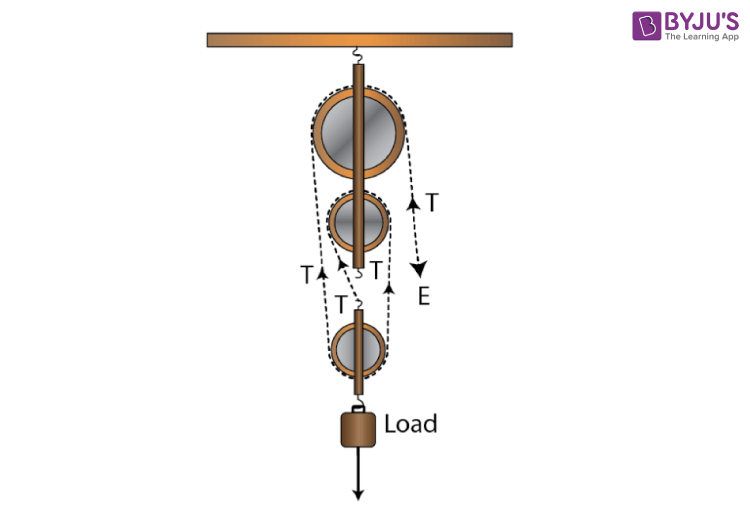(b) The effort put by man is E = 200 kgf

Efficiency η = 60%

For block and tackle system,

η = 1 – w / nE

0.6 = 1 – w / 3 × 200

w / 600 = (1 – 0.6)

Thus W = (1 – 0.6) × 600

w = 240 kgf

L = nE – w

L = 600 – 240

= 360 kgf

(b) The velocity ratio of system is V.R = 3

Therefore, we get

V.R = dE / dL = n = 3

Therefore dL = dE / 3

= 60 / 3

= 20 cm

Question: 11

You are given four pulleys and three strings. Draw a neat and labelled diagram to use them so as to obtain a maximum mechanical advantage equal to 8. In your diagram mark the directions of load L, effort E and tension in each strand.

Solutions: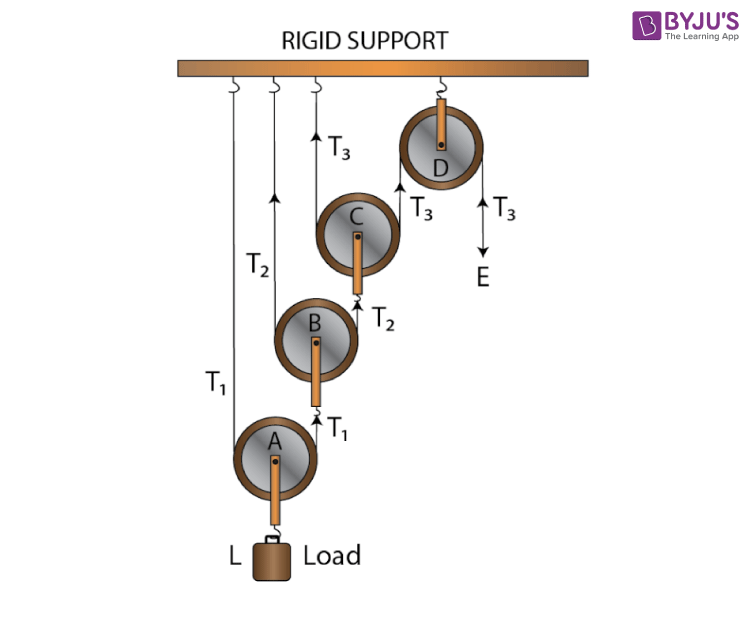Assumptions are

(i) There is no friction in the pulley bearing

(ii) The pulleys and the string are massless

## Selina Solutions Concise Physics Class 10 Chapter 3 Machines

Selina Solutions Concise Physics Class 10 Chapter 3 Machines is related to the study of Machines. Some of the topics which are discussed in this chapter are technical terms related to a machine, principle of a machine, pulley, single fixed pulley. Students clear their doubts completely if they follow Selina Solutions while solving the textbook questions. Frequent practise of these solutions will help students to excel in exams with ease.

List of subtopics covered in Selina Solutions Concise Physics Class 10 Chapter 3 Machines

 Number Subtopic 3.1 Machines 3.2 Technical terms related to a machine 3.3 Principle of a Machine 3.4 Relation between efficiency, mechanical advantage and velocity ratio 3.5 Levers 3.6 Kinds of Levers 3.7 Examples of each class of Levers as found in the human body 3.8 Pulley 3.9 Single fixed pulley 3.10 A single movable pulley 3.11 Combination of pulleys

List of Exercise

 Name of the exercise Page number 3(A) 57 3(B) 66

### Key Features of Selina Solutions Concise Physics Class 10 Chapter 3 Machines

• Selina Solutions are explained in-depth with numerical problems for better understanding
• Subject experts have designed Selina Solutions in a lucid and logical language
• Selina Solutions help the students to build a strong foundation about the concepts covered in this chapter